• 二分图中的关键点是指，若去掉这个点，使得二分图上的最大匹配变小的方案数。 算法 考虑枚举一个点，将它删去后所产生的影响。 设mx(i)mx(i)mx(i)为XXX部中的点所匹配到的YYY部中的点。考虑枚举iii，若mx(i)=−1mx(i...
二分图的关键点
二分图中的关键点是指，若去掉这个点，使得二分图上的最大匹配变小的方案数。
算法
考虑枚举一个点，将它删去后所产生的影响。
设$mx(i)$为$X$部中的点所匹配到的$Y$部中的点。考虑枚举$i$，若$mx(i)=-1$显然这是个非关键点。
否则我们就沿着$i$去找一条增广路，若找到了这么一条增广路，就说明这个点是非关键点。
那么剩下的点就是关键点了。
题目大意
◇题目传送门◆
有$N$个提案，每种提案有一些人支持，每个人也反对一些提案。若一个人所支持的提案被通过了，且他所反对的提案也被否决了，那么他会开心，否则就会沮丧。求如果让所有人都不沮丧必须通过的提案。
分析
考虑将一个人所支持的提案和他所反对的提案的所有者连边，这样我们就得到了一个二分图。
这样我们就可以将这个问题转成求二分图最大独立集问题，可以用总点数 - 最大匹配数求得。
那么我们需要求的就是最大独立集中的关键点的个数。
简单分析一下就是求二分图中非关键点。于是直接套模板。。。
二分图的关键边
算法
同求解二分图的关键点一样，暴力枚举每条边，然后再跑一遍最大匹配，若答案与原先的最大匹配更小，那么这条边就是关键边，于是记录即可。
例题 HDU 1281 棋盘游戏
题目大意
◇题目传送门◆
一个棋盘上只有$K$个位置能够放车。问：

最多能够放上多少辆车使得每行每列仅有一个车；
若有些位置上不放车会使得放上车的数量减少，则称这个位置为关键位置，求棋盘上共有多少个这样的关键位置。

分析
根据二分图的一般套路，我们将每行每列建成一个点，对于能放的位置，我们就在对应的行和列的点上面连一条边就是了。
那么我们跑一遍二分图最大匹配就解决了第一个问题。
考虑第二个问题。若删掉一个位置使得最大匹配变小，即删掉该行该列之间所连的边能使得最大匹配变小，这和关键边的定义是相符的。这样一来这道题就变成了求关键边的模板题。
参考代码
HDU 3517
#include <cstdio>
#include <vector>
#include <cstring>
#include <algorithm>
using namespace std;

const int Maxn = 200;

bool G[Maxn + 5][Maxn + 5];
int matchx[Maxn + 5], matchy[Maxn + 5];
bool vis[Maxn + 5];
bool DFS(int u, int cnty) {
for(int v = 1; v <= cnty; v++) {
if(vis[v] || !G[u][v]) continue;
vis[v] = true;
if(matchy[v] == -1 || DFS(matchy[v], cnty)) {
matchx[u] = v, matchy[v] = u;
return true;
}
}
return false;
}
int find(int cntx, int cnty) {
memset(matchx, -1, sizeof matchx);
memset(matchy, -1, sizeof matchy);
int ret = 0;
for(int i = 1; i <= cntx; i++) {
memset(vis, false, sizeof vis);
if(DFS(i, cnty)) ret++;
}
return ret;
}

int N, A, B;
int like[Maxn + 5];
vector<int> dislike[Maxn + 5];
int X[Maxn + 5], Y[Maxn + 5];

int delx, dely;
bool JudgeX(int u, int cntx) {
for(int i = 1; i <= cntx; i++) {
if(i == delx || vis[i] || !G[i][u])
continue;
vis[i] = true;
if(matchx[i] == -1 || JudgeX(matchx[i], cntx))
return true;
}
return false;
}
bool JudgeY(int u, int cnty) {
for(int i = 1; i <= cnty; i++) {
if(i == dely || vis[i] || !G[u][i])
continue;
vis[i] = true;
if(matchy[i] == -1 || JudgeY(matchy[i], cnty))
return true;
}
return false;
}
bool remainX[Maxn + 5], remainY[Maxn + 5];
vector<int> Solve(int cntx, int cnty) {
memset(remainX, false, sizeof remainX);
memset(remainY, false, sizeof remainY);
for(int i = 1; i <= cntx; i++) {
if(matchx[i] == -1) {
remainX[i] = true;
continue;
}
dely = matchx[i];
memset(vis, false, sizeof vis);
if(JudgeY(i, cnty)) remainY[dely] = true;
}
for(int i = 1; i <= cnty; i++) {
if(matchy[i] == -1) {
remainY[i] = true;
continue;
}
delx = matchy[i];
memset(vis, false, sizeof vis);
if(JudgeX(i, cntx)) remainX[delx] = true;
}
vector<int> ret;
for(int i = 1; i <= cntx; i++)
if(remainX[i]) ret.push_back(like[X[i]]);
for(int i = 1; i <= cnty; i++)
if(remainY[i]) ret.push_back(like[Y[i]]);
sort(ret.begin(), ret.end());
ret.erase(unique(ret.begin(), ret.end()), ret.end());
return ret;
}

void clear() {
memset(G, false, sizeof G);
memset(dislike, 0, sizeof dislike);
}

int main() {
#ifdef LOACL
freopen("in.txt", "r", stdin);
freopen("out.txt", "w", stdout);
#endif
int _;
scanf("%d", &_);
while(_--) {
scanf("%d %d %d", &A, &B, &N);
clear();
int nx = 0, ny = 0;
for(int i = 1; i <= N; i++) {
scanf("%d", &like[i]);
if(like[i] <= A) X[++nx] = i;
else Y[++ny] = i;
int len;
scanf("%d", &len);
while(len--) {
int x;
scanf("%d", &x);
dislike[i].push_back(x);
}
}
for(int i = 1; i <= nx; i++)
for(int j = 1; j <= ny; j++) {
bool flag = false;
for(int k = 0; k < (int)dislike[X[i]].size(); k++)
if(dislike[X[i]][k] == like[Y[j]]) {
flag = true;
break;
}
for(int k = 0; k < (int)dislike[Y[j]].size(); k++)
if(dislike[Y[j]][k] == like[X[i]]) {
flag = true;
break;
}
if(flag) G[i][j] = true;
}
printf("%d\n", N - find(nx, ny));
vector<int> ans = Solve(nx, ny);
printf("%d", ans.size());
for(int i = 0; i < (int)ans.size(); i++)
printf(" %d", ans[i]);
puts("");
}
return 0;
}

HDU 1281
#include <cstdio>
#include <vector>
#include <cstring>
#include <algorithm>
using namespace std;

const int Maxn = 100;

vector<int> G[Maxn + 5];
bool go[Maxn + 5][Maxn + 5];
void addedge(int u, int v) {
G[u].push_back(v);
}

int N, M, K;

inline void clear() {
memset(go, false, sizeof go);
memset(G, 0, sizeof G);
}

bool vis[Maxn + 5];
int match[Maxn + 5];
bool DFS(int u) {
for(int i = 0; i < (int)G[u].size(); i++) {
int v = G[u][i];
if(vis[v] || !go[u][v])
continue;
vis[v] = true;
if(match[v] == -1 || DFS(match[v])) {
match[v] = u;
return true;
}
}
return false;
}
int find() {
int ret = 0;
for(int i = 1; i <= N; i++) {
memset(vis, false, sizeof vis);
if(DFS(i)) ret++;
}
return ret;
}

int main() {
#ifdef LOACL
freopen("in.txt", "r", stdin);
freopen("out.txt", "w", stdout);
#endif
int cnt_case = 1;
while(scanf("%d %d %d", &N, &M, &K) != EOF) {
clear();
for(int i = 1; i <= K; i++) {
int u, v;
scanf("%d %d", &u, &v);
go[u][v] = true;
}
memset(match, -1, sizeof match);
int mx = find(), cnt = 0;
for(int i = 1; i <= N; i++)
for(int j = 1; j <= M; j++) {
if(!go[i][j]) continue;
memset(match, -1, sizeof match);
go[i][j] = false;
if(mx != find()) cnt++;
go[i][j] = true;
}
printf("Board %d have %d important blanks for %d chessmen.\n", cnt_case, cnt, mx);
++cnt_case;
}
return 0;
}



展开全文• 关键点检测基础人脸关键点检测是指给定人脸图像，定位出人脸面部的关键点，包括眉毛、眼睛、鼻子、嘴、脸部轮廓区域的点，由于受到姿态和遮挡等因素的影响，关键点检测是一个富有挑战性的任务。人脸关键点有以下...


点击上方“AI与计算机视觉”，选择加"星标"或“置顶”重磅干货，第一时间送达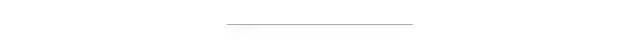人脸关键点检测是诸如人脸识别、表情分析、三维人脸重建等其他人脸相关任务的基础。在人脸识别技术中是人脸检测的下一步任务。关键点检测基础人脸关键点检测是指给定人脸图像，定位出人脸面部的关键点，包括眉毛、眼睛、鼻子、嘴、脸部轮廓区域的点，由于受到姿态和遮挡等因素的影响，关键点检测是一个富有挑战性的任务。人脸关键点有以下主要的应用：人脸姿态对齐、人脸识别等算法都需要对人脸进行特征点检测，实现姿态对齐，从而提高模型的精度。人脸美颜与编辑，基于关键点可以精确分析脸型、眼睛形状、鼻子形状等，从而对人脸的特定位置进行修饰加工，实现人脸的特效美颜、贴片等娱乐功能，也能辅助一些人脸编辑算法更好地发挥作用。人脸表情分析，基于关键点可以对人的面部表情进行分析，从而用于互动娱乐，行为预测等场景。以深度学习的人脸关键点检测：1、模型训练(1)数据集接口数据集接口用于实现图像和标签的获取；class WLFWDatasets(data.Dataset):       def __init__(self, file_list, transforms=None):               self.line = None              self.path = None               self.landmarks = None               self.attribute = None               self.filenames = None               self.euler_angle = None               self.transforms = transforms                # 读取txt文件                with open(file_list, 'r') as f:                        self.lines = f.readlines()       def __getitem__(self, index):                # 每一行存储的分别是图像路径、关键点、属性、欧拉角          self.line = self.lines[index].strip().split()                # 使用opencv读取图像                self.img = cv2.imread(self.line)                # 获得98个关键点                self.landmark = np.asarray(self.line[1:197], dtype=np.float32)               # 获得6个人俩属性                self.attribute = np.asarray(self.line[197:203], dtype=np.int32)               # 获得3个欧拉角                self.euler_angle = np.asarray(self.line[203:206], dtype=np.float32)                if self.transforms:                    # 预处理                        self.img = self.transforms(self.img)                return self.img, self.landmark, self.attribute, self.euler_angle                def __len__(self):                    return len(self.lines)上面代码中，所有的数据和标签都按行存为txt文件，因此需要定义类从txt文件中按行读取图像、关键点、人脸属性、欧拉角。其中关键点为98个，因此维度为196。人脸属性包括6个，分别为：姿态、表情、光照、化妆、遮挡、模糊。(2)模型定义模型包含两个部分，第一部分是关键点回归模型，第二部分是姿态估计模型。首先我们看关键点模型的定义，这是一个基于MobileNet V2的回归模型。它使用了“漏斗形”的残差模块，这是区别于MobileNet V1的基本结构单元。class InvertedResidual(nn.Module):    def __init__(self, inp, oup, stride, use_res_connect, expand_ratio=6):        super(InvertedResidual, self).__init__()        self.stride = stride        # 仅支持1或2两种步长        assert stride in [1, 2]        # 是否使用跳层链接        self.use_res_connect = use_res_connect        self.conv = nn.Sequential(            # 1*1卷积层，通道数从inp升维为inp*expand_ratio            nn.Conv2d(inp, inp * expand_ratio, 1, 1, 0, bias=False),            nn.BatchNorm2d(inp * expand_ratio),            nn.ReLU(inplace=True),            # 3*3分组卷积层            nn.Conv2d(                inp * expand_ratio,                inp * expand_ratio,                3,                stride,                1,                groups=inp * expand_ratio,                bias=False),            nn.BatchNorm2d(inp * expand_ratio),            nn.ReLU(inplace=True),            # 1*1卷积层，通道数从inp*expand_ratio变为oup            nn.Conv2d(inp * expand_ratio, oup, 1, 1, 0, bias=False),            nn.BatchNorm2d(oup),        )    def forward(self, x):        if self.use_res_connect:            return x + self.conv(x)        else:            return self.conv(x)从上面的定义可以看出，“漏斗形”残差块的定义包含3个卷积层，其中，第一个1×1卷积层，实现升维；第二个是3×3的分组卷积；第三个个是1×1卷积层，实现降维。因为中间的纬度高，所以被称为“漏斗形”残差结构。另外，还可以根据变量控制是否使用跳层链接。再看PFLD的模型定义。class PFLDInference(nn.Module):    def __init__(self):        super(PFLDInference, self).__init__()        # 第一个卷积层，输入为图像，输出64个通道，卷积核大小为3，步长为2        self.conv1 = nn.Conv2d(            3, 64, kernel_size=3, stride=2, padding=1, bias=False)        self.bn1 = nn.BatchNorm2d(64)        self.relu = nn.ReLU(inplace=True)        # 第二个卷积层，输入输出都是64通道，卷积核大小3，步长1        self.conv2 = nn.Conv2d(            64, 64, kernel_size=3, stride=1, padding=1, bias=False)        self.bn2 = nn.BatchNorm2d(64)        self.relu = nn.ReLU(inplace=True)        # 堆叠的漏斗形模块        self.conv3_1 = InvertedResidual(64, 64, 2, False, 2)        self.block3_2 = InvertedResidual(64, 64, 1, True, 2)        self.block3_3 = InvertedResidual(64, 64, 1, True, 2)        self.block3_4 = InvertedResidual(64, 64, 1, True, 2)        self.block3_5 = InvertedResidual(64, 64, 1, True, 2)        self.conv4_1 = InvertedResidual(64, 128, 2, False, 2)        self.conv5_1 = InvertedResidual(128, 128, 1, False, 4)        self.block5_2 = InvertedResidual(128, 128, 1, True, 4)        self.block5_3 = InvertedResidual(128, 128, 1, True, 4)        self.block5_4 = InvertedResidual(128, 128, 1, True, 4)        self.block5_5 = InvertedResidual(128, 128, 1, True, 4)        self.block5_6 = InvertedResidual(128, 128, 1, True, 4)        self.conv6_1 = InvertedResidual(128, 16, 1, False, 2)  # [16, 14, 14]                # 卷积层， 输入16通道，输出32通道，卷积核大小3，步长2        self.conv7 = conv_bn(16, 32, 3, 2)  # [32, 7, 7]        # 卷积层， 输入32通道，输出128通道， 卷积核大小7，步长1        self.conv8 = nn.Conv2d(32, 128, 7, 1, 0)  # [128, 1, 1]        self.bn8 = nn.BatchNorm2d(128)                # 两个全局平均池化层        self.avg_pool1 = nn.AvgPool2d(14)        self.avg_pool2 = nn.AvgPool2d(7)                # 全链接层        self.fc = nn.Linear(176, 196)    def forward(self, x):  # x: 3, 112, 112        x = self.relu(self.bn1(self.conv1(x)))  # [64, 56, 56]        x = self.relu(self.bn2(self.conv2(x)))  # [64, 56, 56]        x = self.conv3_1(x)        x = self.block3_2(x)        x = self.block3_3(x)        x = self.block3_4(x)        out1 = self.block3_5(x)        x = self.conv4_1(out1)        x = self.conv5_1(x)        x = self.block5_2(x)        x = self.block5_3(x)        x = self.block5_4(x)        x = self.block5_5(x)        x = self.block5_6(x)                x = self.conv6_1(x)        x1 = self.avg_pool1(x)        x1 = x1.view(x1.size(0), -1)  # 第一个特征，维度16        x = self.conv7(x)        x2 = self.avg_pool2(x)        x2 = x2.view(x2.size(0), -1)  # 第二个特征，维度32        x3 = self.relu(self.conv8(x))        x3 = x3.view(x1.size(0), -1)  # 第三个特征，维度128        multi_scale = torch.cat([x1, x2, x3], 1)  # 多尺度特征融合        landmarks = self.fc(multi_scale)  # 关键点        return out1, landmarks从上面的结构可以看出，首先经过了两个卷积层，然后经过了若干个漏斗形残差模块，最后使用了3个特征尺度进行融合。其中，第一个尺度为14×14，然后进行了全局平均池化，维度是16；第二个尺度为7×7，进行全局平均池化，维度是32；第三个尺度为1×1，维度是128。3个特征变量维度变换后进行了串接，得到总的特征向量维度是176，然后经过全链接层输出196维的关键点坐标landmarks。另一方面，block3_5的输出out1将被作为姿态估计网络输入。最后看看姿态网络估计网络的定义。class AuxiliaryNet(nn.Module):    def __init__(self):        super(AuxiliaryNet, self).__init__()        # 四个卷积层        self.conv1 = conv_bn(64, 128, 3, 2)        self.conv2 = conv_bn(128, 128, 3, 1)        self.conv3 = conv_bn(128, 32, 3, 2)        self.conv4 = conv_bn(32, 128, 7, 1)        # 3×3最大池化层        self.max_pool1 = nn.MaxPool2d(3)        # 两个全链接层        self.fc1 = nn.Linear(128, 32)        self.fc2 = nn.Linear(32, 3)    def forward(self, x):        x = self.conv1(x)        x = self.conv2(x)        x = self.conv3(x)        x = self.conv4(x)        x = self.max_pool1(x)        x = x.view(x.size(0), -1)        x = self.fc1(x)        x = self.fc2(x)        return x可以看出，姿态估计网络包含4个卷积层及1个最大池化层和两个全链接层。其中，第一个卷积层输入是64通道，来自于MobileNet V2的中间特征层，可见两个网络实现了底层特征共享。(3)损失定义class PFLDLoss(nn.Module):    def __init__(self):        super(PFLDLoss, self).__init__()    def forward(self, attribute_gt, landmark_gt, euler_angle_gt, angle, landmarks, train_batchsize):        # 角度权重        weight_angle = torch.sum(1 - torch.cos(angle - euler_angle_gt), axis=1)        # 属性权重        attributes_w_n = attribute_gt[:, 1:6].float()        mat_ratio = torch.mean(attributes_w_n, axis=0)        mat_ratio = torch.Tensor([            1.0 / (x) if x > 0 else train_batchsize for x in mat_ratio        ]).to(device)        weight_attribute = torch.sum(attributes_w_n.mul(mat_ratio), axis=1)        # 欧式距离损失计算        l2_distant = torch.sum((landmark_gt - landmarks) * (landmark_gt - landmarks), axis=1)        return torch.mean(weight_angle * weight_attribute * l2_distant), torch.mean(l2_distant)可以看出，在计算出角度权重和属性权重后就可以结合欧式距离得到最终的损失。2、模型测试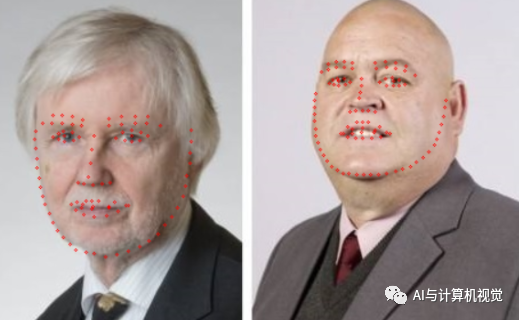训练完成后我们可以看到人脸图像上的关键点检测结果3、总结总体来说，pfld模型对大部分人脸图像有着非常不错的定位结果，对一般姿态和遮挡效果也非常不错，当然也还有比较大的提升空间，包括以下两个方面：增加训练数据集，虽然这里已经是7500张人脸训练的结果，但是对于工业级的人脸关键点检测来说还是远远不够，应该增加年龄、姿态及遮挡类型，从而进一步提高模型的鲁棒性。增加数据增强操作，此处为了验证模型，没有采用在线的数据增强方案，当使用更多的数据增强操作时，比如多尺度训练、颜色扰动和光照变化等，可以提升模型性能。·END·微信号：AI与计算机视觉展开全文• ## 人脸关键点检测总结

万次阅读 多人点赞 2018-05-31 10:38:21
人脸关键点检测也称为人脸关键点检测、定位或者人脸对齐，是指给定人脸图像，定位出人脸面部的关键区域位置，包括眉毛、眼睛、鼻子、嘴巴、脸部轮廓等。 人脸关键点检测方法大致分为三种： - 基于模型的ASM(Active...
概述

人脸关键点检测也称为人脸关键点检测、定位或者人脸对齐，是指给定人脸图像，定位出人脸面部的关键区域位置，包括眉毛、眼睛、鼻子、嘴巴、脸部轮廓等。

人脸关键点检测方法大致分为三种：
- 基于模型的ASM(Active Shape Model)和AAM(Active Appearnce Model)
- 基于深度学习的方法

ASM（Active Shape Model）算法

1. 概要

这是一种1995年提出的很老的关键点检测方法。ASM是基于特征点分布模型（Point Distribution Model,PDM）提取的一种方法。在PDM中，外形相似的物体，例如人脸、人手、心脏、肺部等的几何形状可以通过若干关键特征点（landmarks）的坐标依次串联形成一个形状向量来表示。

ASM跟大多数统计学习方法一样，也包括train和test两部分，也就是形状建模build和形状匹配fit。这个算法其实很简单，可以用来做实时性的检测。

步骤：
==人工标定训练集->对齐构建形状模型->搜索匹配==

2. 特征点标记

为了建立ASM，需要一组标有n个特征点的N幅人脸图象(包括多个人的不同表情和姿态)作为训练数据。特征点可以标记在脸的外部轮廓和器官的边缘，需要注意的是各个标定点的顺序在训练集中的各张照片需要一致。得到特征点集，可以看做一个2n维的向量，n表示特征点数量：
{(x1,y1),(x2,y2),...,(xn,yn)},x=(x1,...,xn,y1,...,yn)T$\left\{\left({x}_{1},{y}_{1}\right),\left({x}_{2},{y}_{2}\right),...,\left({x}_{n},{y}_{n}\right)\right\},x=\left({x}_{1},...,{x}_{n},y1,...,{y}_{n}{\right)}^{T}$
\{(x_1,y_1),(x_2,y_2),...,(x_n,y_n)\}, x=(x_1,...,x_n,y1,...,y_n)^T

3. 模型训练

1）对齐

为了研究训练图象的形状变化，比较不同形状中相对应的点，应先对这些图象进行对齐。

对齐是指以某个形状为基准，对其它形状进行旋转，缩放和平移使其尽可能的与基准形状接近的过程。

首先选择一幅基准图像。训练集中其他图片经过变换尽可能的接近该基准图像，具体的变化过程可以用一个缩放幅度参数s，旋转参数theta以及平移参数矩阵t表示。假设我们要将第2幅图像经过变换尽可能的接近第1幅图像，变换后的图像矩阵表示为：
M(s,θ)[X2]−t$M\left(s,\theta \right)\left[{X}_{2}\right]-t$
M(s,\theta)[X_2]-t

与基准形状尽可能接近，在数学上我们经常用欧式距离的大小衡量接近的程度。假设图像i的标定点矩阵为Xi,图像j的标定点矩阵为Xj,二者的欧式距离大小为
d2ik=(Xi−Xj)T(Xi−Xk)${d}_{ik}^{2}=\left({X}_{i}-{X}_{j}{\right)}^{T}\left({X}_{i}-{X}_{k}\right)$
d_{ik}^2=(X_i-X_j)^T(X_i-X_k)

2） 构建局部特征

对对齐后的形状特征做PCA处理。接着，为每个关键点构建局部特征。目的是在每次迭代搜索过程中每个关键点可以寻找新的位置。局部特征一般用梯度特征，以防光照变化。有的方法沿着边缘的法线方向提取，有的方法在关键点附近的矩形区域提取。

形状搜索

首先：计算眼睛（或者眼睛和嘴巴）的位置，做简单的尺度和旋转变化，对齐人脸；接着，在对齐后的各个点附近搜索，匹配每个局部关键点（常采用马氏距离），得到初步形状；再用平均人脸（形状模型）修正匹配结果；迭代直到收敛。

优点：

1. 得到的特征点是有序；
2. 能根据训练数据对于参数的调节加以限制，从而将形状的改变限制在一个合理的范围内。


缺点：

1. 需要人工对特征点进行顺序标注，工作量超大；
2. 其近似于穷举搜索的关键点定位方式在一定程度上限制了其运算效率。


AAM（Active Appearance Models）算法

1. 概要

1998年，Cootes对ASM进行改进，不仅采用形状约束，而且又加入整个脸部区域的纹理特征，即：Appreance = Shape + Texture，提出了AAM算法。

AAM主动外观模型主要分为两个阶段，模型建立阶段和模型匹配阶段。其中模型建立阶段包括了对训练样本分别建立形状模型(Shape Model)和纹理模型(Texture Model)，然后将两个模型进行结合，形成AAM模型。模型匹配阶段是指在视频序列中将已建立好的AAM模型在当前帧图像中寻找最匹配的目标的过程。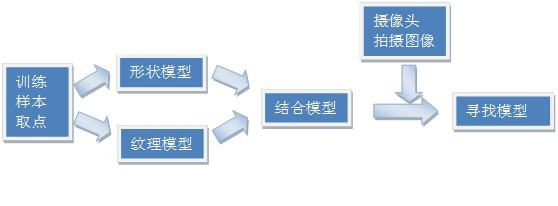2. 样本选取与标定

1）要建立AAM模型，就需要采集目标的样本，建立样本库。
2）要建立关于人脸的AAM模型，就需要把目标从样本中提取出来而去除背景等的影响。而提取目标的方法就是在样本中手动把人脸的轮廓标定出来。
3）在手动标定了样本中的点后，通过程序把样本的点的位置{x,y}保存为向量的形式

3. 形状模型（shape model）

对输入数据首先建立活动形状模型ASM。建立ASM模型包括Procrustes变换和PCA降维两个步骤。Procrustes变换就是ASM中的对齐操作。样本和形状模型的建立步骤与ASM相同。

4. 纹理模型（Texture Model）

纹理模型(Texture Model)描述了目标的纹理特征。纹理模型的输入数据和形状模型一样，在这里可以看做是n张图片，每张图片上有一个点集。
1）Delaunay三角划分：将空间点连接为三角形，使得所有三角形中最小的角最大的一个技术。三角划分的要点是任何三角形的外接圆都不包括任何其他顶点，即外接圆性质。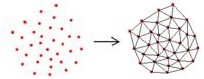2）三角映射：使用Delaunay三角划分后，每一个样本都可以划分为一系列三角形，而不同样本间这些三角形是对应的。要得到平均纹理模型，就需要把样本中的目标的纹理通过分片仿射投影到同一个样本上，而这种分片仿射就是三角映射。

先设定一个样本为平均脸，既然在样本中的点与平均脸的所有三角形都是一一对应的，就可以根据三角形中每一点的位置计算其对应平均脸中某一点的位置，然后把该点的像素值复制到平均脸对应点的位置上。最终每一个样本都可以转化到平均脸上，然后把这n个脸求平均，就得到了平均纹理模型。

5. 组合模型（Combine Model）

在得到形状模型和纹理模型后，我们就可以对这两个模型进行加权组合，得到组合模型，也就是AAM模型。同时我们也得到了p+q个参数，再降维得到r个参数，这些参数就是用来改变AAM模型的特征的。

6. 搜索模型（Search Model）

AAM模型建立以后，就可以实际在视频序列中跟踪人脸。

初始时记AAM模型的r个参数为向量，然后对输入的图像，首先假设某个位置有人脸，得到人脸的实际参数c,然后我们通过不断地调整使c和r之间的距离最小，若这个最小值小于某个阀值，就可以认为当前位置出现人脸，我们就能够在视频中检测到了人脸。优点：

1. 与ASM相比有增加了纹理信息
2. 模型简单直接，架构清晰明确，易于理解和应用


缺点：

1. 需要人工对特征点进行顺序标注，工作量超大；
2. 对噪声非常敏感。


1. 概要

2. 回归器训练

人脸关键点检测的目的是估计向量，K表示关键点的个数，由于每个关键点有横纵两个坐标，所以S的长度为2K。

在每个阶段中首先进行特征提取，这里使用的是shape-indexed features，也可以使用诸如HOG、SIFT等人工设计的特征，或者其他可学习特征（learning based features）。

姿态θ训练过程是先初始化一个θ，然后提取特征，再用特征进行回归，再更新θ。然后通过训练得到的回归器R来估计增量ΔS( update vector)，把ΔS加到前一个阶段的S上得到新的S，这样通过不断的迭代即可以得到最终的S(shape)。加上训练R的损失函数:

L=∑i=1Nd(θTi,θi)$L=\sum _{i=1}^{N}d\left({\theta }_{i}^{T},{\theta }_{i}\right)$
L=\sum^N_{i=1}{d(\theta_i^T,\theta_i)}

作者用到的回归方法为Random Fern Regressors，这种算法思想类似于随机森林。级联回归器的训练过程为：根据θ的不断更新，关键位置的预测结果如图3. 姿势聚类

用不同的初始姿态θ训练级联分类器会得到不同的预测结果，有时候初始化姿态选的不合适就会导致错误预测。所以作者对每一个图像，初始化K个不同姿态，CPR运行K次得到K个不同结果。对这些结果进行聚类，选取密度最高的结果作为最终结果。

4. 人脸关键点

作者用了Caltech人脸数据集，对三个关键点即双眼和嘴巴进行位置预测。优点：

1. 算法思想类似于随机森林回归，是一种回归算法，结构清晰简单。
2. 可以用较少的训练数据训练出较好的模型、


缺点：

1. 只对人脸的3个关键点进行检查；
2. 特征提取方式很传统，且作者并没有明确说人脸回归方法预测的准确度。


Face Alignment at 3000 FPS via Regressing Local Binary Features（LBF）

1. 概要

CVPR14年的文章，这篇论文主要讲：Face Alignment 问题,即给人脸确定位置68个标点 (landmark)。而这些标点位置肯定是最能区别不同人的位置。

https://github.com/FacialLandmark/landmark_py

2. 算法步骤

算法步骤可以分为三个过程：
1）提取特征(shape index feature)
2）LBF编码(learning local binary feature)
3）获取shape 增量(learning global linear regression)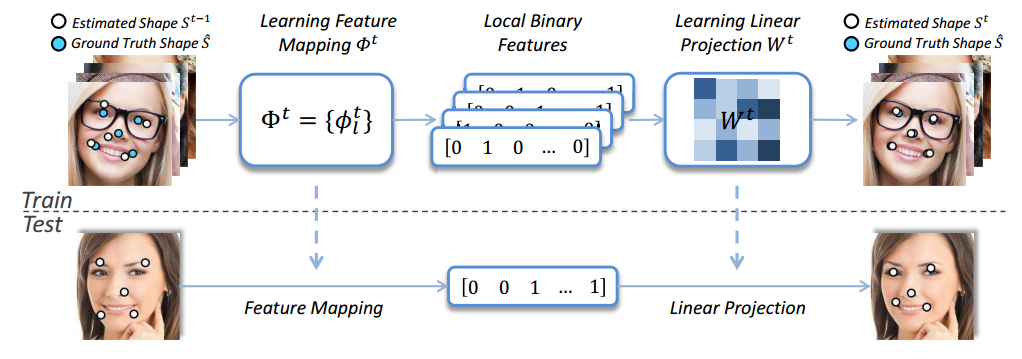训练过程中，先初始化一个shape St-1，用一种特征映射方法Φt生成local binary features。根据特征和目标shape的增量生成最终shape。权值通过线性回归进行更新。在测试阶段，直接预测形状增量，并应用于更新当前估计的形状。形状增量：
ΔSt=WtΦt(I,St−1)$\mathrm{\Delta }{S}^{t}={W}^{t}{\mathrm{\Phi }}^{t}\left(I,{S}^{t-1}\right)$
\Delta S^t =W^t\Phi^t(I,S^{t-1})

3. 提取shape index feature

shape index feature 也就是特征和现有的一系列标定点是相关的，3000fps的做法是在landmark周围随机出两个偏移量，形成两个点，用这两个点的像素差作为特征。

刚开始的时候，shape和ground truth差距太大，因此需要取样特征的半径大一些，最后的shape和ground truth已经很接近了，半径要小一些。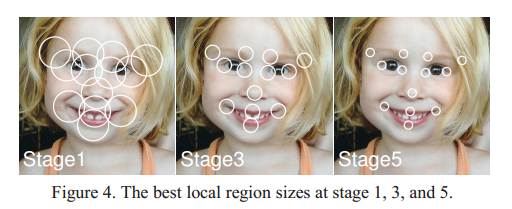4. 学习local binary features

使用标准随机森林训练Φt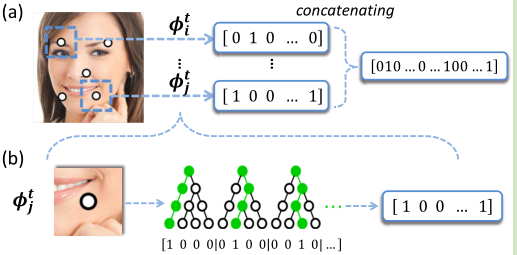(b)所示是标点j用随机森林得到二进制特征，再串联成(a),组成全局特征，最后训练全局线性投影Wt.

随机森林的思想：随机是随机抽取n对像素，这n个像素就成为一个模板(template),以后一直不变。再比较每一对像素，第一个像素大于第二个就为1，第二个大于第一个就为0，再串联n对像素比较值，组成二进制向量就为随机森林特征。如图取的是到达叶子节点为1，道理一样（大于节点遍历左子节点，小于遍历右子节点).

5. 权值学习

使用的双坐标下降方法，使下公式最小：
minWt∑i=1N||ΔSti−WtΦt(Ii,St−1i)||22+λ||Wt||22$\underset{{W}^{t}}{min}\sum _{i=1}^{N}||\mathrm{\Delta }{S}_{i}^{t}-{W}^{t}{\mathrm{\Phi }}^{t}\left({I}_{i},{S}_{i}^{t-1}\right)|{|}_{2}^{2}+\lambda ||{W}^{t}|{|}_{2}^{2}$
\min_{W^t}\sum^N_{i=1}||\Delta S^t_i-W^t\Phi^t(I_i,S_i^{t-1})||_2^2+\lambda||W^t||_2^2

λ是控制Wt长度，即让Wt尽量稀疏。

测试的时候，先对一幅图像提取shape index feature, 然后使用随机森林进行编码，最后使用w估计shape 增量。

优点：

1. 速度快，在笔记本上跑了3000FPS，智能手机是300FPS
2. 是最先进算法（the state of the art)有更高的精确性，做为人脸验证底层实现，实时性显然重要。


缺点：

1. 只对人脸的3个关键点进行检查；
2. 参数很难调。


Joint Cascade Face Detection and Alignment

1. 概要

这篇是MSRA在14年发表在ECCV上的论文，这篇文章直接在30ms的时间里把detection和alignment都给做了，PR曲线彪到很高，时效性高，内存占用却非常低。这篇文章的思想继承了CPR，使用级联树+简单特征的结构，将分类与回归都完成，计算简单,高效。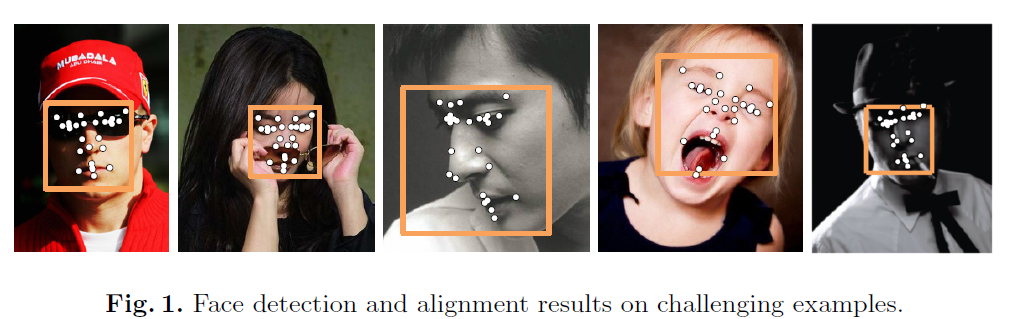文章的思想是训练一棵决策树同时做Face Alignment和Face Detection。

2. Post classifier

在人脸对齐前需要先将人脸检测出来。

1）样本准备：首先作者调用opencv的Viola-Jones分类器，将recall阀值设到99%，这样能够尽可能地检测出所有的脸，但是同时也会有非常多的不是脸的东东被检测出来。于是，检测出来的框框们被分成了两类：是脸和不是脸。这些图片被resize到96*96。

2）特征提取：接下来是特征提取，怎么提取呢？作者采用了三种方法：

第一种：把window划分成6*6个小windows，分别提取SIFT特征，然后连接着36个sift特征向量成为图像的特征。

第二种：先求出一个固定的脸的平均shape（27个特征点的位置，比如眼睛左边，嘴唇右边等等），然后以这27个特征点为中心提取sift特征，然后连接后作为特征。

第三种：用他们组去年的另一个成果Face Alignment at 3000 FPS via Regressing Local Binary Features (CVPR14) ，也就是图中的3000FPS方法，回归出每张脸的shape，然后再以每张脸自己的27个shape points为中心做sift，然后连接得到特征。


3）分类：将上述的三种特征分别扔到线性SVM中做分类，训练出一个能分辨一张图是不是脸的SVM模型。紧接着作者将以上三种方法做出的分类器和初始分类器进行比对，画了一个样本分布的图：这个图从左到右依次是原始级联分类器得到的样本分类分布和第一种到第三种方法提取的特征得到的样本分类分布。可见做一下shape alignment可以得到一个更好的分类效果。但是效率很渣，recall 99%一张图可以检测出3000个框。

3. 级联分类器

和随机森林很相似，级联分类器就是一种bagging的思想。定义形状S为一个2L维的向量，L是标点总数。逐步回归的过程是：
St=St−1+Rt(x,St−1),t=1,...,T${S}^{t}={S}^{t-1}+{R}^{t}\left(x,{S}^{t-1}\right),t=1,...,T$
S^t=S^{t-1}+R^t(x,S^{t-1}),t=1,...,T

每一个Rt是一个回归函数，它会在前一级形状的基础上增加一个形状增量。学习时使当前形状St与真实形状S之间的误差最小。

一个关键的创新点在于，在级联标点框架中，每一个回归器Rt依赖于前一个形状S(t-1),在训练学习的过程中，特征定义为与S(t-1)相关，所以称之为姿态/形状索引特征，这种特征对面部形状变化时，呈现出很好的几何不变性。

将这种姿态/形状索引特征同样的应用在检测环节,则文章统一两个训练学习任务，将分类器定义为：
f=∑t=1T∑k=1KCtk(x,St−1)$f=\sum _{t=1}^{T}\sum _{k=1}^{K}{C}_{k}^{t}\left(x,{S}^{t-1}\right)$
f=\sum^T_{t=1}\sum^K_{k=1}C_k^t(x,S^{t-1})

这样的分类器相当于结合了形状标定S和分类检测C。算法过程训练结果：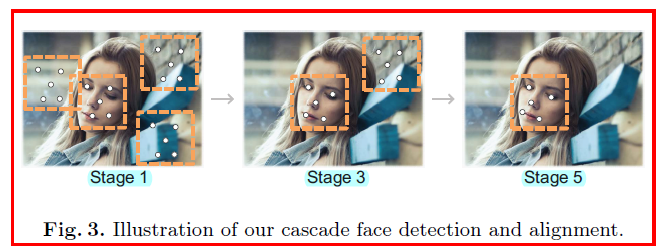4. 实验效果

使用像素差作为特征使得高性能得到保证。算法在VGA图像（640*480pixel）上的检测性能是28.6ms/张。检测器只占用15MB内存。在FDDB数据集上。算法的Recall为80.07%。
优点：

1. 速度快，占用内存小。
2. 可以同时做人脸和人脸关键点检测


缺点：

1. 参数难调。


One Millisecond Face Alignment with an Ensemble of Regression Trees（ETR）

1. 概要

CVPR 2014的一篇关于人脸关键点检测的论文，基于Ensemble of Regression Tress算法(以下简称 ERT)，速度极快（单人人脸关键点检测耗时约为1ms），效果也不错。同时又能处理训练集中部分关键点标定缺失的情况。LBF（Face Alignment at 3000 FPS via Regressing Local Binary Features）这篇论文的进阶版本。

2. 算法思想

LBF是基于Tree的方法，学习每个关键点的局部二值特征，然后将特征组合起来，使用线性回归检测关键点。与 LBF 不同的是， ERT 是在学习 Tree的过程中，直接将 shape 的更新值 ΔS存入叶子结点 leaf node. 初始位置 S 在通过所有学习到的 Tree后，mean shape 加上所有经过的叶子结点的ΔS，即可得到最终的人脸关键点位置。总体流程如下图所示：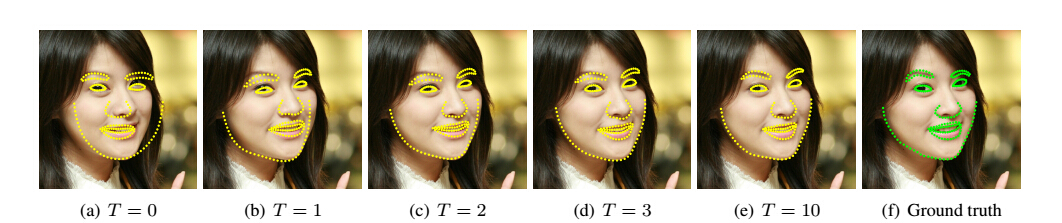公式表达是：
St+1=St+1+rt(I,St)${S}^{t+1}={S}^{t+1}+{r}_{t}\left(I,{S}^{t}\right)$
S^{t+1}=S^{t+1}+r_t(I,S^t)

其中 t 表示级联序号，rt(∙,∙)表示当前级的回归器regressor。回归器的输入参数为图像 I 和上一级回归器更新后的 shape, 采用的特征可以是灰度值或者其它。

为了训练每一级的 rt，文章采用了 gradient tree boosting算法减小 initial shape 和 ground truth 的平方误差总和。

每个回归器由很多棵树(tree)组成，每棵树参数是根据 current shape 和 ground truth 的坐标差和随机挑选的像素对训练得到的。

优点：

1. 速度快，占用内存小；
2. 改进了参数难调的问题。


缺点：

1. 模型稍大（随机数算法的通病）。


Deep Convolutional Network Cascade for Facial Point Detection

1. 概要

2013年，Sun等人首次将CNN应用到人脸关键点检测，提出一种级联的CNN（拥有三个层级）——DCNN(Deep Convolutional Network)，此种方法属于级联回归方法。作者通过设计拥有三个层级的级联卷积神经网络对面部5个关键点左眼、右眼、鼻子、嘴巴两侧进行检测。

2. 整体结构

如图所示，DCNN由三个Level构成。Level-1 由3个CNN组成；Level-2由10个CNN组成（每个关键点采用两个CNN）；Level-3同样由10个CNN组成。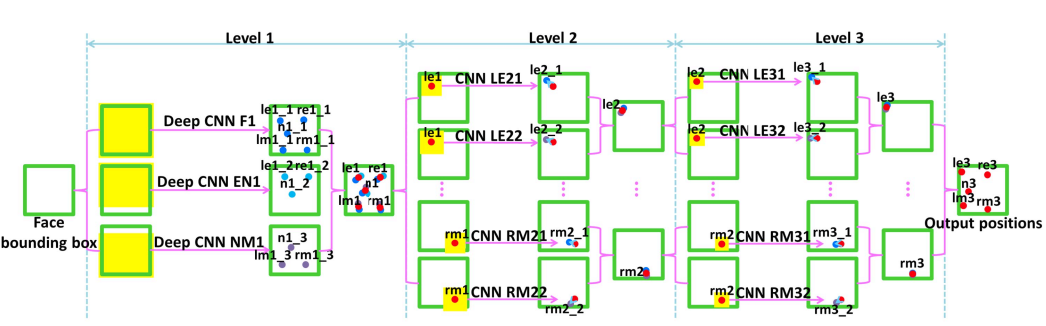1）Level-1分3个CNN，分别是F1（Face1）、EN1（Eye，Nose）、NM1（Nose，Mouth）；F1输入尺寸为39*39，输出5个关键点的坐标；EN1输入尺寸为39*31，输出是3个关键点的坐标；NM11输入尺寸为39*31，输出是3个关键点。Level-1的输出是由三个CNN输出取平均得到。

2）Level-2，由10个CNN构成，输入尺寸均为15*15，每两个组成一对，一对CNN对一个关键点进行预测，预测结果同样是采取平均。

3）Level-3与Level-2一样，由10个CNN构成，输入尺寸均为15*15，每两个组成一对。Level-2和Level-3是对Level-1得到的粗定位进行微调，得到精细的关键点定位。

Level-1之所以比Level-2和Level-3的输入要大，是因为作者认为，由于人脸检测器的原因，边界框的相对位置可能会在大范围内变化，再加上面部姿态的变化，最终导致输入图像的多样性，因此在Level-1应该需要有足够大的输入尺寸。Level-1与Level-2和Level-3还有一点不同之处在于，Level-1采用的是局部权值共享（Locally Sharing Weights）

3. 网络结构

第一级的网络深度要深，分析3个选择网络的重要因素。讨论集中在最难训练的第一级网络。

1）第一级的网络必须要深。从大的输入区域中预测关键点是一个高级任务。更深的结构有利于形成全局的高级特征，在低层，由于局部感受野，神经元提取的特征是局部的。通过结合空间上相邻的低层特征，高层的神经元能从更大的区域提取特征。此外，高层的特征是高度非线性的，增加额外的层增强了从输入到输出的非线性，更有可能代表输入和输出的关系。

2）第二，对卷积层上的神经元，在双曲正切激活函数后的绝对值校正(下一章)能有效提高效果。

3）第三，局部地共享权值有利于更好的表现。

多级回归

由于脸部检测器的不稳定性和姿态的多样性。所以第一级的输入区域应该是足够大来覆盖所有可能的预测。但大的输入区域是主要的不准确原因，因为不相关的区域可能退化网络最后的输出。

第一级的网络输出为接下来的检测提供了一个强大的先验知识。真实的脸部点伪装分布在第一级预测的一个小领域内。所以第二级的检测可以在一个小范围内完成。但没有上下文信息，局部区域的表现是不可靠的。为了避免发散，我们不能级联太多层，或者过多信任接下来的层。这些网络只能在一个小范围内调整初始预测。

为了更好的提高检测精度和可靠性，作者提出了每一级都有多个网络共同地预测每一个点。这些网络的不同在于输入区域。最后的预测可以用公式表达如下：

x=x(1)1+...+x(1)l1l1+∑i=2nΔx(i)1+...+x(i)l1li$x=\frac{{x}_{1}^{\left(1\right)}+...+{x}_{{l}_{1}}^{\left(1\right)}}{{l}_{1}}+\sum _{i=2}^{n}\frac{\mathrm{\Delta }{x}_{1}^{\left(i\right)}+...+{x}_{{l}_{1}}^{\left(i\right)}}{{l}_{i}}$
x=\frac{x_1^{(1)}+...+x_{l_1}^{(1)}}{l_1}+\sum_{i=2}^n\frac{\Delta x_1^{(i)}+...+x_{l_1}^{(i)}}{l_i}

优点：

1. 改善初始不当导致陷入局部最优的问题；
2. 借助于CNN强大的特征提取能力，获得更为精准的关键点检测。


缺点：

1. 只有5个关键点检测
2. 3.3GHz的CPU单张图片检测速度0.12s，网络结构复杂。


Extensive Facial Landmark Localization with Coarse-to-Fine Convolutional Network Cascade

这篇是ICCV2013年face++在DCNN模型上改进提出的从粗到精的人脸关键点检测算法。实现了68个人脸关键点的高精度定位。该算法将人脸关键点分为内部关键点和轮廓关键点，内部关键点包含眉毛、眼睛、鼻子、嘴巴共计51个关键点，轮廓关键点包含17个关键点。

整体结构

网络采用四级级联结构，与上一篇文章相似。本篇最大的创新点在于：在网络的输入方面，不是用人脸检测器检测到的人脸区域图片作为网络的输入，而是采用CNN预测人脸的bounding box，这个改进对初始level定位精度提高非常多。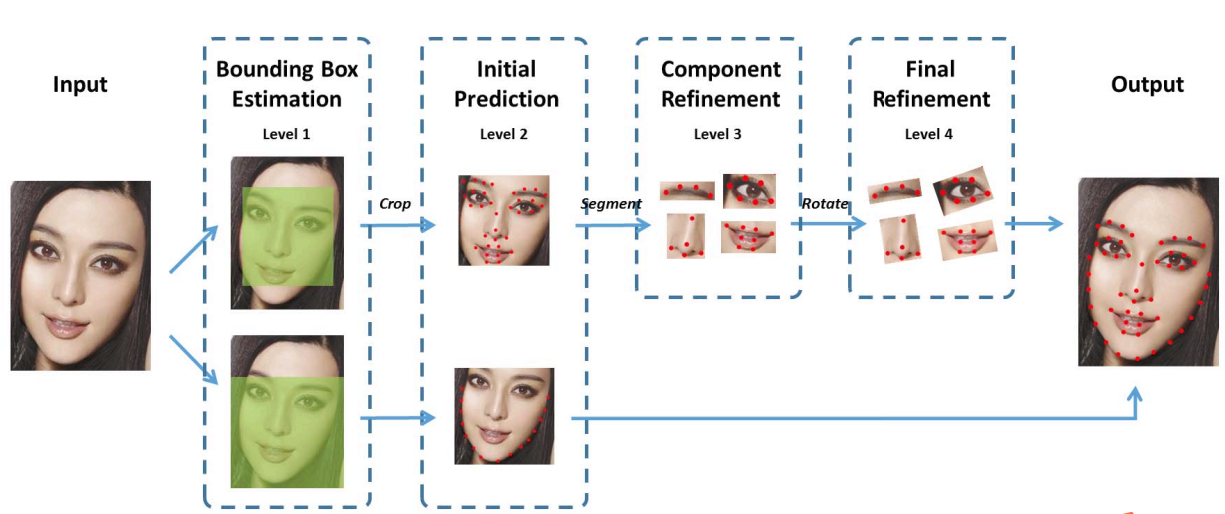1）Level-1主要作用是获得面部器官的边界框，上面是inner的bounding box，下面是contour的bounding box；

2）Level-2的输出是51个关键点预测位置，这里起到一个粗定位作用，目的是为了给Level-3进行初始化；

3）Level-3会依据不同器官进行从粗到精的定位；

4）Level-4的输入是将Level-3输出的inner point进行一定的旋转，最终将51个关键点的位置进行输出。

针对外部17个关键点contour point，仅采用两个层级的级联网络进行检测。

网络结构

N1表示为inner point各个特征点的初始位置预测，也就是相当于inner 的level 2。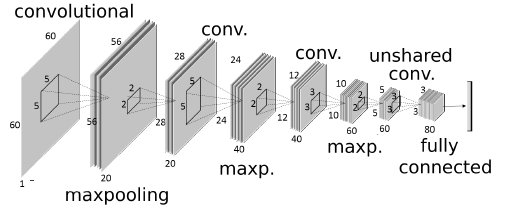网络结构方面基本上与传统的CNN模型，差不多，最大的区别在于locally sharing weights，也就是上面的unshared conv层，我们平时所遇到的CNN都是global sharing weights的。但是对于特征点的预测，五官的高层特征差别比较大，所以需要在高层采用unshared weight的方式，在低层采用global sharing weights。

优点：

1. 把人脸的关键点定位问题，划分为内部关键点和轮廓关键点分开预测，有效的避免了loss不均衡问题；
2. 内部关键点检测部分，并未像DCNN那样每个关键点采用两个CNN进行预测，而是每个器官采用一个CNN进行预测，从而减少计算量。


缺点：

1. 模型多，运算复杂。


Facial Landmark Detection by Deep Multi-task Learning（TCDCN）

1. 概述

这篇文章发表于ECCV2014，来自香港中文大学汤晓鸥课题组。

文章提出了人脸特征点检测的新方法，使用与人脸相关的属性共同学习人脸的特征点位置。具体而言，就是在人脸特征点检测的时候，同时进行多个任务的学习，这些任务包括：性别，是否带眼镜，是否微笑和脸部的姿势。使用这些辅助的属性帮助更好的定位特征点，根据论文结果，这样的确对人脸特征点检测有一定的帮助。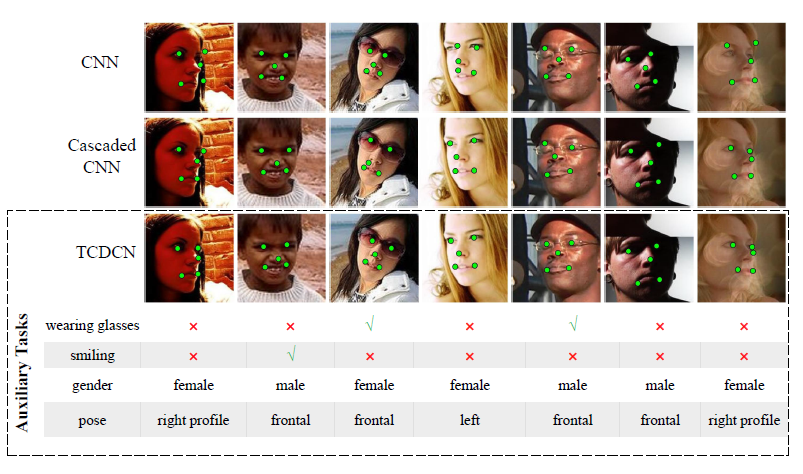https://github.com/zhzhanp/TCDCN-face-alignment
https://github.com/flyingzhao/tfTCDCN

2. 多任务检测模型

argminw∑t=1T∑i=1Nl(yti,f(xti;wt))+ϕ(wt)$argmi{n}_{w}\sum _{t=1}^{T}\sum _{i=1}^{N}l\left({y}_{i}^{t},f\left({x}_{i}^{t};{w}^{t}\right)\right)+\varphi \left({w}^{t}\right)$
argmin_w\sum_{t=1}^T\sum_{i=1}^Nl(y_i^t,f(x_i^t;w^t))+\phi(w^t)

损失函数就是不同任务的损失函数直接相加。而在人脸特征点检测的任务中，不同的任务具有不同的loss，特征点检测是平方和误差，而其它分类任务是交叉熵误差，因此最后的loss就是：
argminwt,wa12∑i=1N||yri−f(xi;Wr)||2−∑i=1N∑a∈Aλayailog(p(yai|xi;Wa))+∑t=1T||W||22$argmi{n}_{{w}^{t},{w}^{a}}\frac{1}{2}\sum _{i=1}^{N}||{y}_{i}^{r}-f\left({x}_{i};{W}^{r}\right)|{|}^{2}-\sum _{i=1}^{N}\sum _{a\in A}{\lambda }^{a}{y}_{i}^{a}log\left(p\left({y}_{i}^{a}|{x}_{i};{W}^{a}\right)\right)+\sum _{t=1}^{T}||W|{|}_{2}^{2}$
argmin_{w^t,w^a}\frac{1}{2}\sum_{i=1}^N||y_i^r-f(x_i;W^r)||^2-\sum_{i=1}^N\sum_{a\in A}\lambda^ay_i^alog(p(y_i^a|x_i;W^a))+\sum_{t=1}^T||W||^2_2

从这个式子可以看到，损失函数的前部分就是特征点检测，后部分是不同的分类任务loss相加，最后一项是正则项。在训练时，各个任务使用相同的特征，只有在最后一级才根据任务的不同做不同处理（线性回归或者分类）。

3. 网络结构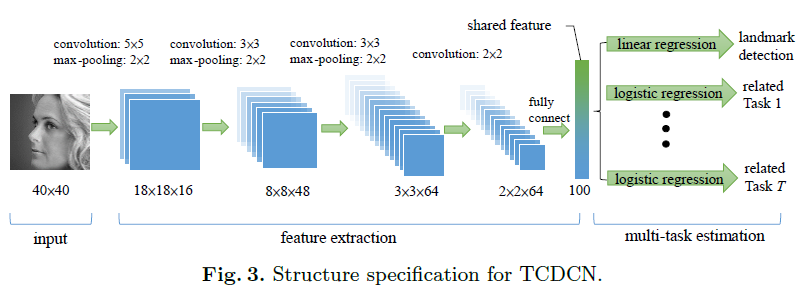输入图片为40*40的黑白图片，然后经过4个卷积层变成2*2*64的图像，其中激活函数采用|tanh|，即对tanh取绝对值。在最后的全连接层将2*2*64的多层结构变成100个shared feature，以上部分都可以看成特征提取的操作。在最后一层由这些特征进行不同操作，对特征点检测问题就是linear regression，对于其他分类问题就是logistic regression（softmax）。

针对多任务学习的特点，本文提出了一种新的early stopping方法。当辅助任务达到最好表现以后，这个任务就对主要任务没有帮助了，就可以停止这个任务。如下式所示，Eaval是任务a的验证集loss，Eatr是训练集loss，如果下面式子超过了某个阈值ϵ就会停止这个任务。
k⋅medtj=t−kEatr(j)∑tj=t−kEatr(j)−k⋅medtj=t−kEatr(j)⋅Eaval(t)−minj=1..tEatr(j)λa⋅minj=1..tEatr(j)>ϵ$\frac{k\cdot me{d}_{j=t-k}^{t}{E}_{t}^{a}r\left(j\right)}{\sum _{j=t-k}^{t}{E}_{tr}^{a}\left(j\right)-k\cdot me{d}_{j=t-k}^{t}{E}_{tr}^{a}\left(j\right)}\cdot \frac{{E}_{val}^{a}\left(t\right)-\underset{j=1..t{E}_{tr}^{a}\left(j\right)}{min}}{{\lambda }^{a}\cdot \underset{j=1..t}{min}{E}_{tr}^{a}\left(j\right)}>ϵ$
\frac{k\cdot med^t_{j=t-k}E^a_tr(j)}{\sum_{j=t-k}^tE^a_{tr}(j)-k\cdot med_{j=t-k}^tE_{tr}^a(j)} \cdot \frac{E^a_{val}(t)-\min_{j=1..tE_{tr}^a(j)}}{\lambda^a\cdot \min_{j=1..t}E^a_{tr}(j)}>\epsilon

优点：

1. 采用多任务学习方法对人脸关键点进行检测；
2. 对比于级联CNN方法，在Intel Core i5 cpu上，级联CNN需要0.12s，而TCDCN仅需要17ms，速度提升七倍有余。


缺点：

1. 论文中很很多超参数需要调整。


1. 概述

https://github.com/xiangrufan/keras-mtcnn

2. 网络结构

MTCNN包含三个级联的多任务卷积神经网络，分别是Proposal Network (P-Net)、Refine Network (R-Net)、Output Network (O-Net)，每个多任务卷积神经网络均有三个学习任务，分别是人脸分类、边框回归和关键点定位。网络结构如图所示：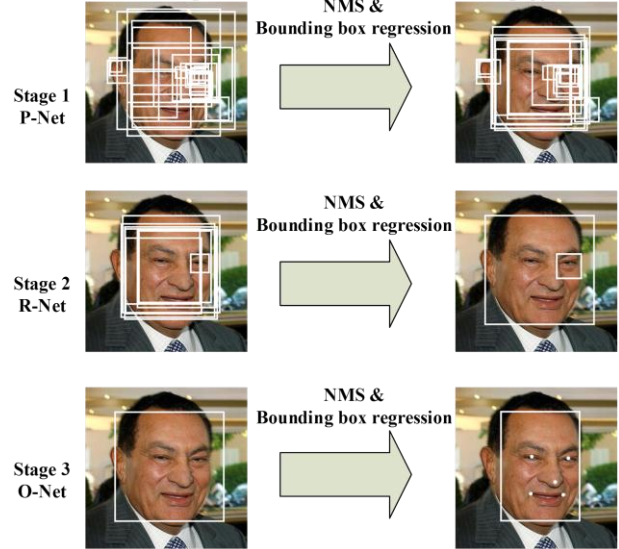TCNN实现人脸检测和关键点定位分为三个阶段。

1）第一阶段，通过一个浅层的CNN快速生成候选窗口。该阶段是一个全部由卷积层组成的CNN，取名P-Net，获取候选人脸窗口以及人脸框回归向量。基于人脸框回归向量对候选窗口进行校正。之后采用NMS合并高重叠率的候选窗口。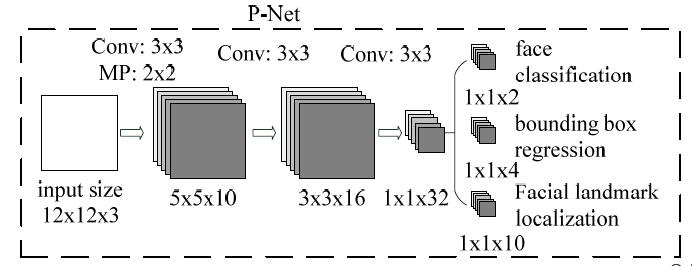2）第二阶段，通过一个更复杂的CNN否决大量非人脸窗口从而精化人脸窗口。第一阶段输出的候选窗口作为R-Net的输入，R-Net能够进一步筛除大量错误的候选窗口，再利用人脸框回归向量对候选窗口做校正，并执行NMS。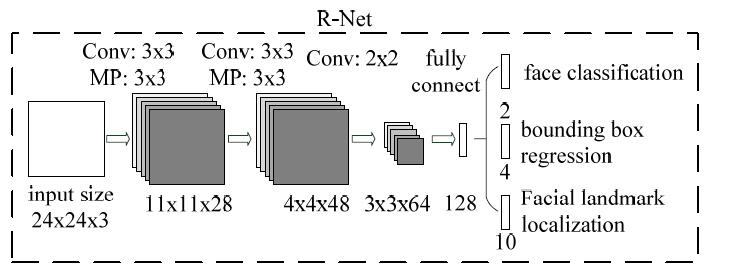3）使用更复杂的CNN进一步精化结果并输出5个人脸特征点。与stage2相似，但这一阶段用更多的监督来识别人脸区域，而且网络能够输出五个人脸特征点位置坐标。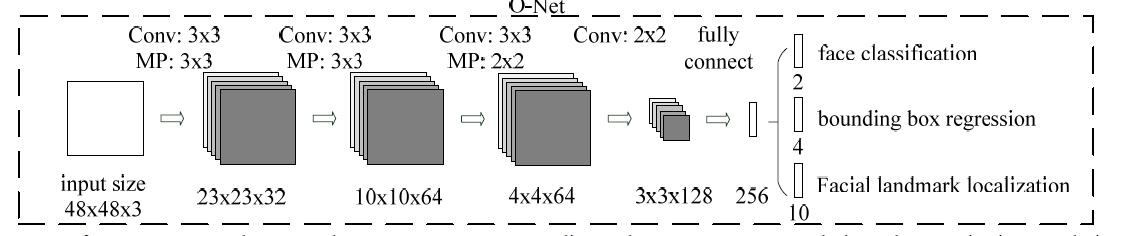3. 训练阶段

在具体训练过程中，作者就多任务学习的损失函数计算方式进行相应改进。在多任务学习中，当不同类型的训练图像输入到网络时，有些任务时是不进行学习的，因此相应的损失应为0。例如，当训练图像为背景（Non-face）时，边界框和关键点的loss应为0，文中提供计算公式自动确定loss的选取，公式为：
min∑i=1N∑jαjβjiLji,j∈{det,box,landmark}$min\sum _{i=1}^{N}\sum _{j}{\alpha }_{j}{\beta }_{i}^{j}{L}_{i}^{j},j\in \left\{det,box,landmark\right\}$
min\sum_{i=1}^N\sum_j{\alpha_j\beta_i^jL_i^j},{j\in \{det,box,landmark\}}

其中， αj表示任务的重要程度，在P-Net和R-Net中，αdet=1,αbox=0.5,αlandmark=0.5 ,在O-Net中，由于要对关键点进行检测，所以相应的增大任务的重要性，αdet=1,αbox=0.5 , αlandmark=1 。 作为样本类型指示器。

实验结果表明，MTCNN在人脸检测数据集FDDB 和WIDER FACE以及人脸关键点定位数据集LFPW均获得当时最佳成绩。在运行时间方面，采用2.60GHz的CPU可以达到16fps，采用Nvidia Titan Black可达99fps

优点：

1. 采用CNN方法同时预测人脸和人脸关键点；
2. 对于速度和精度都有一定的提升。


缺点：

1. 网络结构较复杂。


Facial Landmark Detection with Tweaked Convolutional Neural Networks（TCNN)

1. 概述

2016年，Wu等人研究了CNN在人脸关键点定位任务中到底学习到的是什么样的特征，在采用GMM（Gaussian Mixture Model, 混合高斯模型）对不同层的特征进行聚类分析，发现网络进行的是层次的，由粗到精的特征定位，越深层提取到的特征越能反应出人脸关键点的位置。针对这一发现，提出了TCNN（Tweaked Convolutional Neural Networks）。

https://github.com/flyingzhao/mxnet_VanillaCNN

2. 网络结构

其网络结构如图所示：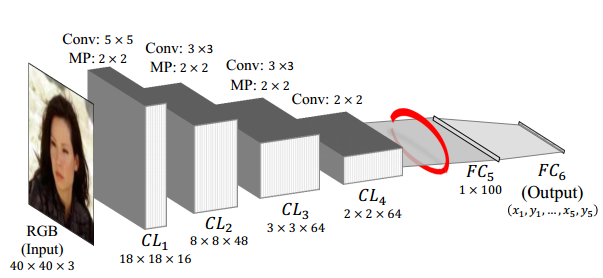图为Vanilla CNN，针对FC5得到的特征进行K个类别聚类，将训练图像按照所分类别进行划分，用以训练所对应的FC6K 。测试时，图片首先经过Vanilla CNN提取特征，即FC5的输出。将FC5输出的特征与K个聚类中心进行比较，将FC5输出的特征划分至相应的类别中，然后选择与之相应的FC6进行连接，最终得到输出。

3. 特征聚类

作者对Vanilla CNN中间各层特征进行聚类分析，并统计出关键点在各层之间的变化程度，如图所示：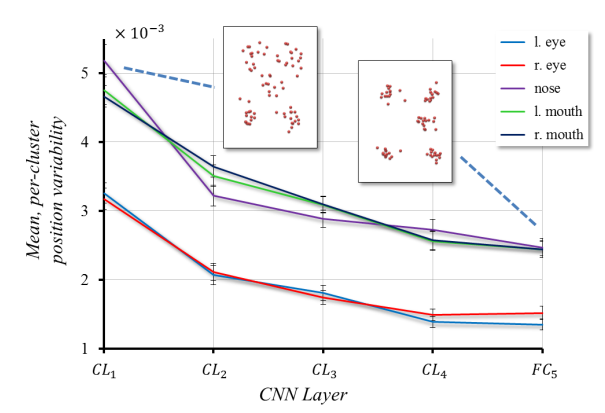从图中可知，越深层提取到的特征越紧密，因此越深层提取到的特征越能反应出人脸关键点的位置。作者在采用K=64时，对所划分簇的样本进行平均后绘图如下：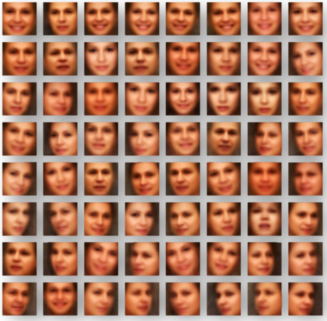从图上可发现，每一个簇的样本反应了头部的某种姿态，甚至出现了表情和性别的差异。因此可推知，人脸关键点的位置常常和人脸的属性相关联。因此为了得到更准确的关键点定位，作者使用具有相似特征的图片训练对应的回归器。网络结构修改为FC5不同的类别对应不同的FC6。FC6用回归的方法对人脸的5个关键点进行检测。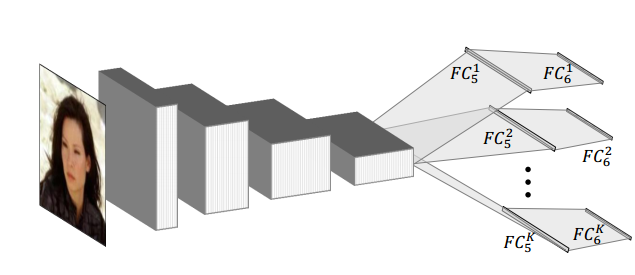优点：

1. 对CNN提取的特征进行聚类，将各簇对应的样本进行分析，最后发现同一簇表现出“相同属性”（姿态，微笑，性别）的人脸。对此，设计了K个FC5和K个FC6层，用以对不同“面部属性”的人脸进行关键点检测。


缺点：

1. TCNN相当于按照姿态的不同，细分了K个“子模型”，只不过K个“子模型”共用卷积层用以提取特征，然后对应的有自己的FC5，FC6层。感觉这个模型的鲁棒性和泛化性不太好。


DAN-Deep Alignment Network: A convolutional neural network for robust face alignment

1. 概述

DAN-Deep Alignment Network，发表于CVPR-2017。以往级联神经网络输入的是图像的某一部分，与以往不同，DAN各阶段网络的输入均为整张图片。当网络均采用整张图片作为输入时，DAN可以有效的克服头部姿态以及初始化带来的问题，从而得到更好的检测效果。之所以DAN能将整张图片作为输入，是因为其加入了关键点热图（Landmark Heatmaps），关键点热图的使用是本文的主要创新点。
https://github.com/MarekKowalski/DeepAlignmentNetwork

2. 网络结构

DAN基本框架如图所示：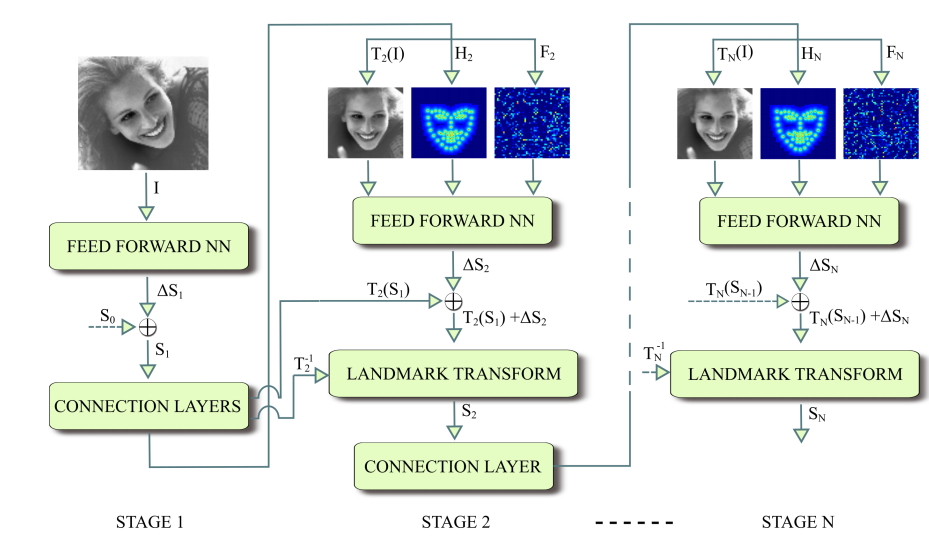DAN包含多个阶段，每一个阶段含三个输入和一个输出，输入分别是被矫正过的图片、关键点热图和由全连接层生成的特征图，输出是面部形状（Face Shape）。其中，CONNECTION LAYER的作用是将本阶段得输出进行一系列变换，生成下一阶段所需要的三个输入，具体操作如下图所示：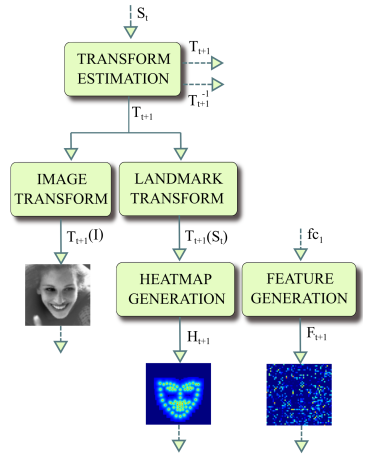第一阶段的输入仅有原始图片和S0。面部关键点的初始化即为S0，S0是由所有关键点取平均得到，第一阶段输出S1。对于第二阶段，首先，S1经第一阶段的CONNECTION LAYERS进行转换，分别得到转换后图片T2（I）、S1所对应的热图H2和第一阶段fc1层输出，这三个正是第二阶段的输入。如此周而复始，直到最后一个阶段输出SN。文中给出在数据集IBUG上，经过第一阶段后的T2（I）、T2（S1）和特征图，如图所示：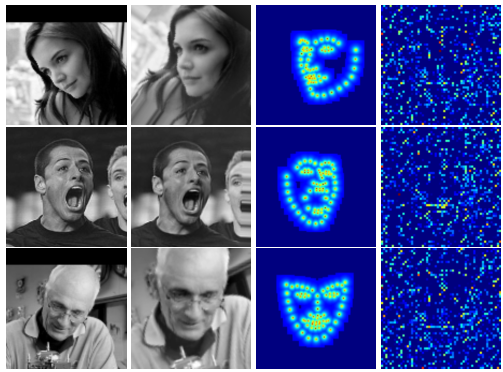3. 前馈神经网络

前馈神经网络的网络结构如图，其输出为landmark的偏移量，这里与以往用回归方法求偏移不同，这里用神经网络输出计算shape的偏移。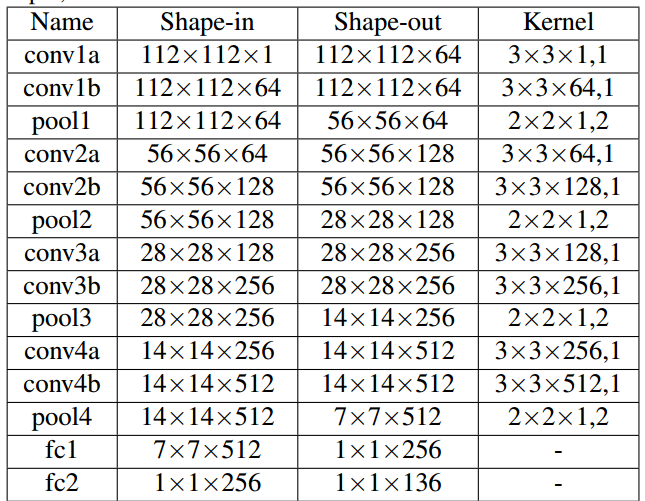DAN要做的“变换”，就是把图片给矫正了，DAN对姿态变换具有很好的适应能力，或许就得益于这个“变换”。St是如何由St-1以及该阶段CNN得到，先看St计算公式：
St=T−1t(Tt(St−1)+ΔSt)${S}_{t}={T}_{t}^{-1}\left({T}_{t}\left({S}_{t-1}\right)+\mathrm{\Delta }{S}_{t}\right)$
S_t=T^{-1}_t(T_t(S_{t-1})+\Delta S_t)

该CNN的输入均是经过了“变换”Tt的操作，因此得到的偏移量 ΔSt是在新特征空间下的偏移量，在经过偏移之后应经过一个反变换T−1t 还原到原始空间。而这里提到的新特征空间，或许是将图像进行了“矫正”，使得网络更好的处理图像。

4. Landmark热力图

关键点热度图的计算就是一个中心衰减，关键点处值最大，越远则值越小，公式如下：
H(x,y)=11+minsi∈Tt(St−1)||(x,y)−si||$H\left(x,y\right)=\frac{1}{1+mi{n}_{{s}_{i}\in {T}_{t}\left({S}_{t-1}\right)}||\left(x,y\right)-{s}_{i}||}$
H(x,y)=\frac{1}{1+min_{s_i\in T_t(S_{t-1})}||(x,y)-s_i||}

优点：

1. DAN是一个级联思想的关键点检测方法；
2. 通过引入关键点热图作为补充，DAN可以从整张图片进行提取特征，从而获得更为精确的定位。


缺点：

1. 网络结构很复杂，还需要计算heatmap；
2. 在1070 GPU上单张图片检测为73fps，连续图片检测为45fps。


How far are we from solving the 2D & 3D Face Alignment problem?

概述

ICCV 2017的论文，结合最先进的人脸特征点定位（landmark localization）架构和最先进的残差模块（residual block），首次构建了一个非常强大的基准，在一个超大2D人脸特征点数据集（facial landmark dataset）上训练，并在所有其他人脸特征点数据集上进行评估。2D-FAN结构

通过堆叠四个HG（HourGlass）构建的人脸对齐网络（Face Alignment Network, FAN），其中所有的 bottleneck blocks（图中矩形块）被替换为新的分层、并行和多尺度block。本质上来说就是一个小卷积网络的4层堆叠来预测人脸。输入2D图片，输出2D图片的Heatmap。

2D 检测结果：2D-to-3D-FAN网络架构

文章首先构建人脸对齐网络“FAN”（Face Alignment Network），然后基于FAN，构建2D-to-3D-FAN，也即将给定图像2D面部标注转换为3D的网络。文章表示，据测试所知，在大规模2D/3D人脸对齐实验中训练且评估FAN这样强大的网络，还尚属首次。

基于人体姿态估计架构HourGlass，输入是RGB图像和2D图片的heatmaps，输出是对应的3D Heatmaps。3D检测结果：优点：

1. 提出了一种新的FAN网络结构来预测人脸关键点；
2. 实现了2D到3D关键点的一种转换。


缺点：

1. 3D的检测结果还是不够准确；
2. 文章工作还包括标注3D数据集，所以技术细节提及不多。


OpenFace: A general-purpose face recognition library with mobile applications

1. 概述

OpenFace是很热门的人脸检测和人脸对齐开源库，这个pipeline可以看做是使用深度卷积网络处理人脸问题的一个基本框架，她结合了Dlib和传统的决策树人脸对齐方法。

https://github.com/cmusatyalab/openface

2. 基本结构

它的结构如下图所示：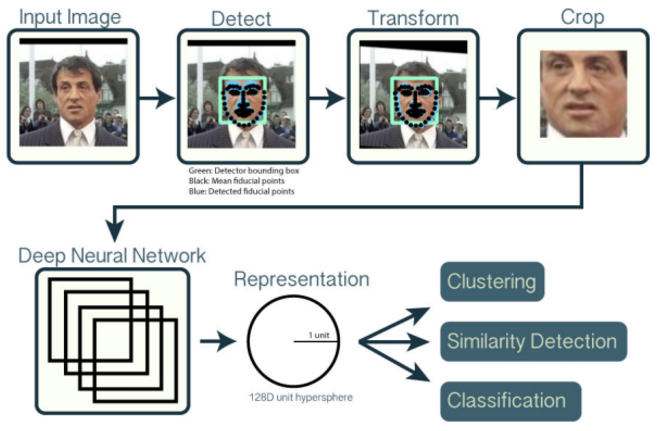1）Input Image -> Detect
输入：原始的可能含有人脸的图像。
输出：人脸位置的bounding box。

这一步一般我们称之为“人脸检测”（Face Detection），在OpenFace中，使用的是dlib、OpenCV现有的人脸检测方法。此方法与深度学习无关，使用的特征是传统计算机视觉中的方法（一般是Hog、Haar等特征）。

2）Detect -> Transform -> Crop
输入：原始图像 + 人脸位置bounding box
输出：“校准”过的只含有人脸的图像

对于输入的原始图像 + bounding box，这一步要做的事情就是要检测人脸中的关键点，然后根据这些关键点对人脸做对齐校准。所谓关键点，就是下图所示的绿色的点，通常是眼角的位置、鼻子的位置、脸的轮廓点等等。有了这些关键点后，我们就可以把人脸“校准”，或者说是“对齐”。解释就是原先人脸可能比较歪，这里根据关键点，使用仿射变换将人脸统一“摆正”，尽量去消除姿势不同带来的误差。这一步我们一般叫Face Alignment。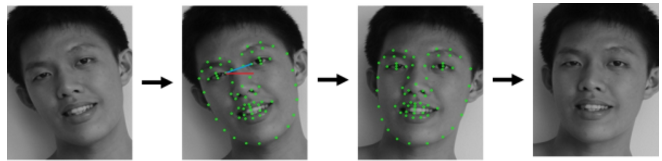在OpenFace中，这一步同样使用的是传统方法，特点是比较快，对应的论文是： 《One Millisecond Face Alignment with an Ensemble of Regression Trees》

3）Crop -> Representation
输入：校准后的单张人脸图像
输出：一个向量表示。

这一步就是使用深度卷积网络，将输入的人脸图像，转换成一个向量的表示。在OpenFace中使用的向量是128x1的，也就是一个128维的向量。
优点：

1. openface相当于是做了一个整合，把人脸检测，人脸对齐方法结合了一下。
2. 前面的检测和对齐用到的是传统方法，所以速度比较快。


缺点：

1. 只是做了整合，神经网络实际只是用于后续的人脸分类。

展开全文• OpenCV基于OpenPose的手部关键点检测概述✔️ 手部关键点检测，旨在找出给定图片中手指上的关节点及指尖关节点, 其中手部关键点检测的应用场景主要包括：手势...手部关键点检测器的实现主要基于论文：Hand Keypoi...OpenCV基于OpenPose的手部关键点检测概述✔️ 手部关键点检测，旨在找出给定图片中手指上的关节点及指尖关节点, 其中手部关键点检测的应用场景主要包括：手势识别手语识别与理解手部的行为识别✔️ Opencv的DNN手部识别主要基于 CMU Perceptual Computing Lab 开源的手部关键点检测模型OpenPose。手部关键点检测器的实现主要是基于论文：Hand Keypoint Detection in Single Images using Multiview Bootstrapping - CVPR2017其中，如下：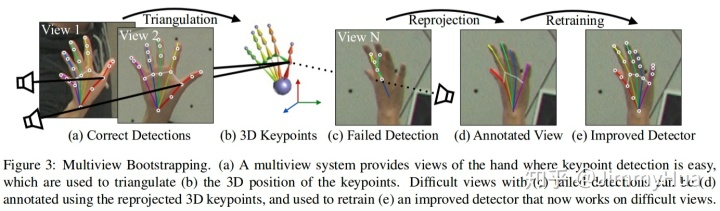✏️ 论文中，首先采用少量标注的人手部关键点图像数据集，训练类似于人体姿态关键点所使用的CPM - Convolutional Pose Machines 网络，以得到手部关键点的粗略估计. 采用了 31个 HD 高清摄像头从不同的视角对人手部进行拍摄。然后，将拍摄图像送入手部关键点检测器，以初步得到许多粗略的关键点检测结果。一旦有了同一手部的不同视角的关键点，则构建关键点测量(Keypoint triangulation)，以得到关键点的3D位置。关键点的3D位置被从3D重新投影到每一幅不同视角的 2D 图片，并采用2D图像和关键点，进一步训练网络，以鲁棒的预测手部关键点位置，这对于关键点难以预测的图片而言是尤其重要的。采用这种方式，通过少量几次迭代，即可得到较为准确的手部关键点检测器.⛳ 总之，关键点检测器和多视角图像(multi-view images) 一起构建了较为准确的手部关键点检测模型. 采用的检测网络类似于人体关键点中所用的网络结构. 进度提升的主要因素是采用了多视角图片标注图片数据集.✔️ 手部关键点检测模型共输出 22 个关键点，其中包括手部的 21 个点，第 22 个点表示背景. 如图：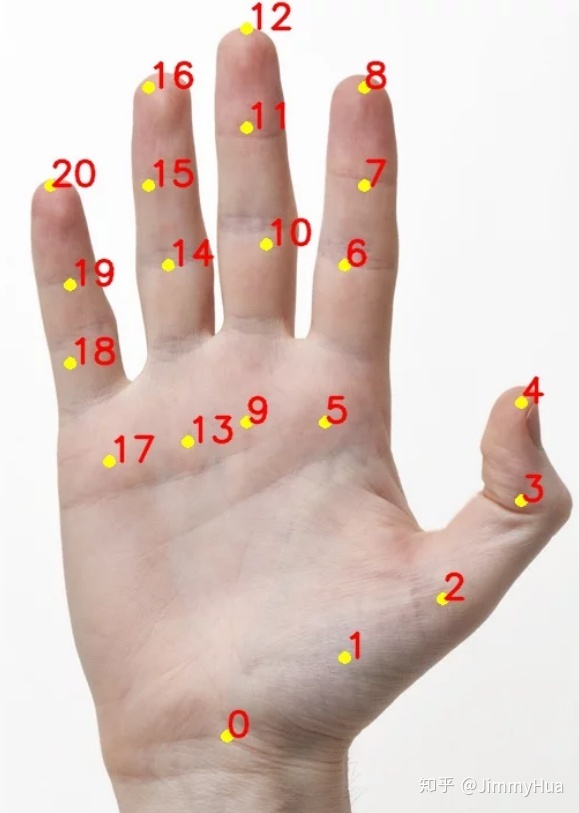手部关键点代码示例☑️️ ️模型文件准备： - hand/pose_deploy.prototxt - hand/pose_iter_102000.caffemodel☑️️ python代码：import os
import cv2
import time
import numpy as np
import matplotlib.pyplot as plt

class general_pose_model(object):
def __init__(self, modelpath):
self.num_points = 22
self.point_pairs = [[0,1],[1,2],[2,3],[3,4],
[0,5],[5,6],[6,7],[7,8],
[0,9],[9,10],[10,11],[11,12],
[0,13],[13,14],[14,15],[15,16],
[0,17],[17,18],[18,19],[19,20]]
self.inHeight = 368
self.threshold = 0.1
self.hand_net = self.get_hand_model(modelpath)

# 模型加载
def get_hand_model(self, modelpath):

prototxt   = os.path.join(modelpath, "pose_deploy.prototxt")
caffemodel = os.path.join(modelpath, "../pose_iter_102000.caffemodel")

return hand_model

# 预测
def predict(self, imgfile):
img_height, img_width, _ = img_cv2.shape
aspect_ratio = img_width / img_height

inWidth = int(((aspect_ratio * self.inHeight) * 8) // 8)
inpBlob = cv2.dnn.blobFromImage(img_cv2, 1.0 / 255, (inWidth, self.inHeight), (0, 0, 0), swapRB=False, crop=False)

self.hand_net.setInput(inpBlob)

output = self.hand_net.forward()

# vis heatmaps
self.vis_heatmaps(imgfile, output)

points = []
for idx in range(self.num_points):
probMap = output[0, idx, :, :] # confidence map.
probMap = cv2.resize(probMap, (img_width, img_height))

# Find global maxima of the probMap.
minVal, prob, minLoc, point = cv2.minMaxLoc(probMap)

if prob > self.threshold:
points.append((int(point), int(point)))
else:
points.append(None)

return points

# heatmap可视化
def vis_heatmaps(self, imgfile, net_outputs):
plt.figure(figsize=[10, 10])

for pdx in range(self.num_points):
probMap = net_outputs[0, pdx, :, :]
probMap = cv2.resize(probMap, (img_cv2.shape, img_cv2.shape))
plt.subplot(5, 5, pdx+1)
plt.imshow(cv2.cvtColor(img_cv2, cv2.COLOR_BGR2RGB))
plt.imshow(probMap, alpha=0.6)

plt.colorbar()
plt.axis("off")
plt.show()

# 手部关键点可视化
def vis_pose(self, imgfile, points):
img_cv2_copy = np.copy(img_cv2)
for idx in range(len(points)):
if points[idx]:
cv2.circle(img_cv2_copy, points[idx], 8, (0, 255, 255), thickness=-1,
lineType=cv2.FILLED)
cv2.putText(img_cv2_copy, "{}".format(idx), points[idx], cv2.FONT_HERSHEY_SIMPLEX,
1, (0, 0, 0), 2, lineType=cv2.LINE_AA)

# 绘制连接点
for pair in self.point_pairs:
partA = pair
partB = pair

if points[partA] and points[partB]:
cv2.line(img_cv2, points[partA], points[partB], (0, 255, 255), 3)
cv2.circle(img_cv2, points[partA], 8, (0, 0, 255), thickness=-1, lineType=cv2.FILLED)

plt.figure(figsize=[10, 10])

plt.subplot(1, 2, 1)
plt.imshow(cv2.cvtColor(img_cv2, cv2.COLOR_BGR2RGB))
plt.axis("off")
plt.subplot(1, 2, 2)
plt.imshow(cv2.cvtColor(img_cv2_copy, cv2.COLOR_BGR2RGB))
plt.axis("off")
plt.show()

if __name__ == '__main__':
print("[INFO]Pose estimation.")
os.listdir(imgs_path)]
img_files = ['hand.jpg']
start = time.time()
modelpath = ""
pose_model = general_pose_model(modelpath)
print("[INFO]Model loads time: ", time.time() - start)

for img_file in img_files:
start = time.time()
res_points = pose_model.predict(img_file)
print("[INFO]Model predicts time: ", time.time() - start)
pose_model.vis_pose(img_file, res_points)

print("[INFO]Done.") - 输出heatmap：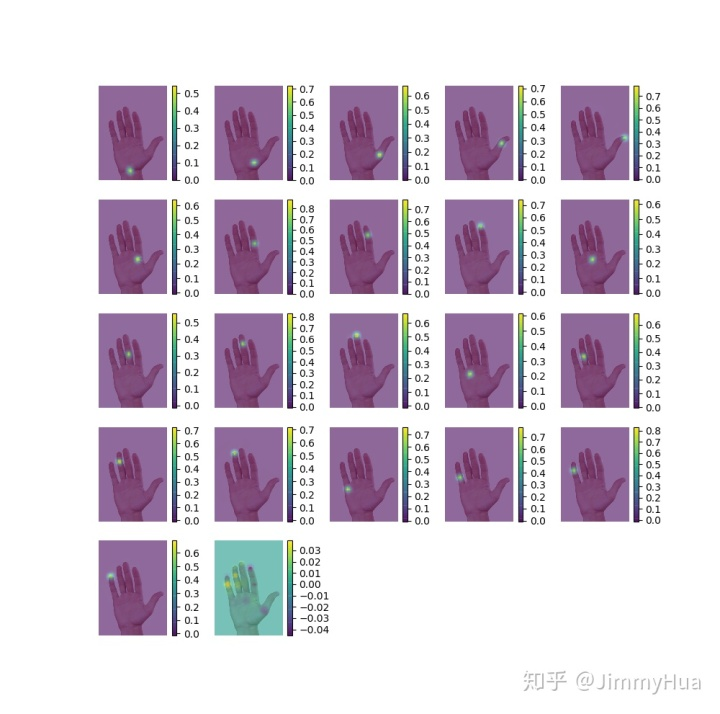- 输出关键点：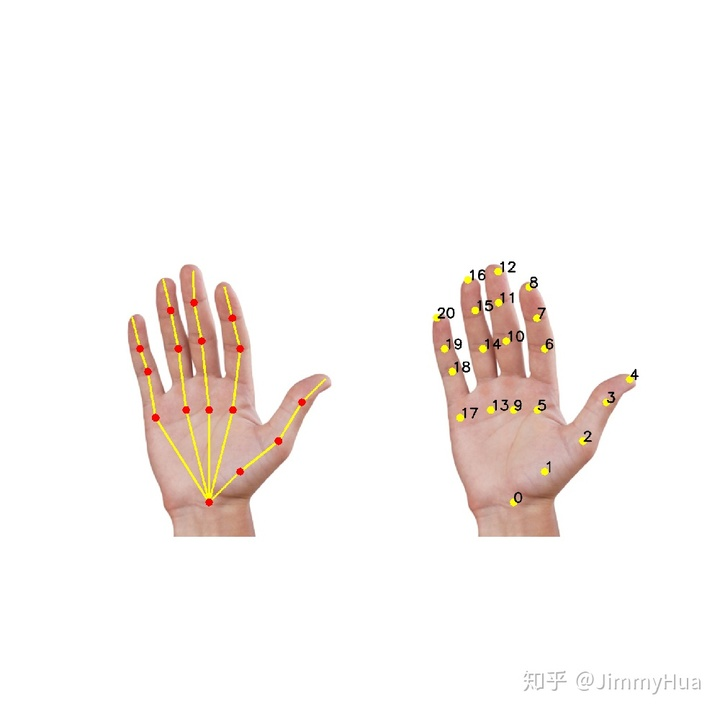OpenCV于OpenPose的人体姿态估计✔️ 同样，Opencv也可以结合OpenPose进行人体姿态估计，具体实现和手部关键点检测类似，只是调用的模型函数有所区别，具体代码实现可以参考下文。☑️ 模型文件下载：OpenPose 人体姿态模型下载路径：BODY25: http://posefs1.perception.cs.cmu.edu/OpenPose/models/pose/body_25/pose_iter_584000.caffemodel
COCO: http://posefs1.perception.cs.cmu.edu/OpenPose/models/pose/coco/pose_iter_440000.caffemodel
MPI: http://posefs1.perception.cs.cmu.edu/OpenPose/models/pose/mpi/pose_iter_160000.caffemodel

COCO prototxt：https://github.com/CMU-Perceptual-Computing-Lab/openpose/blob/master/models/pose/coco/pose_deploy_linevec.prototxt☑️ 代码实现：import cv2
import time
import numpy as np
import matplotlib.pyplot as plt
import os

class general_pose_model(object):
def __init__(self, modelpath, mode="BODY25"):
# 指定采用的模型
#   Body25: 25 points
#   COCO:   18 points
#   MPI:    15 points
self.inWidth = 368
self.inHeight = 368
self.threshold = 0.1
if mode == "BODY25":
self.pose_net = self.general_body25_model(modelpath)
elif mode == "COCO":
self.pose_net = self.general_coco_model(modelpath)
elif mode == "MPI":
self.pose_net = self.get_mpi_model(modelpath)

def get_mpi_model(self, modelpath):
self.points_name = {
"RShoulder": 2, "RElbow": 3, "RWrist": 4,
"LShoulder": 5, "LElbow": 6, "LWrist":
7, "RHip": 8, "RKnee": 9, "RAnkle": 10,
"LHip": 11, "LKnee": 12, "LAnkle": 13,
"Chest": 14, "Background": 15 }
self.num_points = 15
self.point_pairs = [[0, 1], [1, 2], [2, 3],
[3, 4], [1, 5], [5, 6],
[6, 7], [1, 14],[14, 8],
[8, 9], [9, 10], [14, 11],
[11, 12], [12, 13]
]
prototxt = os.path.join(
modelpath,
"pose/mpi/pose_deploy_linevec_faster_4_stages.prototxt")
caffemodel = os.path.join(
modelpath,
"pose/mpi/pose_iter_160000.caffemodel")

return mpi_model

def general_coco_model(self, modelpath):
self.points_name = {
"Nose": 0, "Neck": 1,
"RShoulder": 2, "RElbow": 3, "RWrist": 4,
"LShoulder": 5, "LElbow": 6, "LWrist": 7,
"RHip": 8, "RKnee": 9, "RAnkle": 10,
"LHip": 11, "LKnee": 12, "LAnkle": 13,
"REye": 14, "LEye": 15,
"REar": 16, "LEar": 17,
"Background": 18}
self.num_points = 18
self.point_pairs = [[1, 0], [1, 2], [1, 5],
[2, 3], [3, 4], [5, 6],
[6, 7], [1, 8], [8, 9],
[9, 10], [1, 11], [11, 12],
[12, 13], [0, 14], [0, 15],
[14, 16], [15, 17]]
prototxt   = os.path.join(
modelpath,
"openpose_pose_coco.prototxt")
caffemodel = os.path.join(
modelpath,
"../pose_iter_440000.caffemodel")
print(prototxt, caffemodel)

return coco_model

def general_body25_model(self, modelpath):
self.num_points = 25
self.point_pairs = [[1, 0], [1, 2], [1, 5],
[2, 3], [3, 4], [5, 6],
[6, 7], [0, 15], [15, 17],
[0, 16], [16, 18], [1, 8],
[8, 9], [9, 10], [10, 11],
[11, 22], [22, 23], [11, 24],
[8, 12], [12, 13], [13, 14],
[14, 19], [19, 20], [14, 21]]
prototxt   = os.path.join(
modelpath,
"pose/body_25/pose_deploy.prototxt")
caffemodel = os.path.join(
modelpath,
"pose/body_25/pose_iter_584000.caffemodel")

return coco_model

def predict(self, imgfile):
img_height, img_width, _ = img_cv2.shape
inpBlob = cv2.dnn.blobFromImage(img_cv2,
1.0 / 255,
(self.inWidth, self.inHeight),
(0, 0, 0),
swapRB=False,
crop=False)
self.pose_net.setInput(inpBlob)
self.pose_net.setPreferableBackend(cv2.dnn.DNN_BACKEND_OPENCV)
self.pose_net.setPreferableTarget(cv2.dnn.DNN_TARGET_OPENCL)

output = self.pose_net.forward()

H = output.shape
W = output.shape
print(output.shape)

# vis heatmaps
self.vis_heatmaps(img_file, output)

#
points = []
for idx in range(self.num_points):
probMap = output[0, idx, :, :] # confidence map.

# Find global maxima of the probMap.
minVal, prob, minLoc, point = cv2.minMaxLoc(probMap)

# Scale the point to fit on the original image
x = (img_width * point) / W
y = (img_height * point) / H

if prob > self.threshold:
points.append((int(x), int(y)))
else:
points.append(None)

return points

def vis_heatmaps(self, imgfile, net_outputs):
plt.figure(figsize=[10, 10])

for pdx in range(self.num_points):
probMap = net_outputs[0, pdx, :, :]
probMap = cv2.resize(
probMap,
(img_cv2.shape, img_cv2.shape)
)
plt.subplot(5, 5, pdx+1)
plt.imshow(cv2.cvtColor(img_cv2, cv2.COLOR_BGR2RGB))
plt.imshow(probMap, alpha=0.6)
plt.colorbar()
plt.axis("off")
plt.show()

def vis_pose(self, imgfile, points):
img_cv2_copy = np.copy(img_cv2)
for idx in range(len(points)):
if points[idx]:
cv2.circle(img_cv2_copy,
points[idx],
3,
(0, 0, 255),
thickness=-1,
lineType=cv2.FILLED)
cv2.putText(img_cv2_copy,
"{}".format(idx),
points[idx],
cv2.FONT_HERSHEY_SIMPLEX,
.6,
(0, 255, 255),
1,
lineType=cv2.LINE_AA)

# Draw Skeleton
for pair in self.point_pairs:
partA = pair
partB = pair

if points[partA] and points[partB]:
cv2.line(img_cv2,
points[partA],
points[partB],
(0, 255, 0), 3)
cv2.circle(img_cv2,
points[partA],
3,
(0, 0, 255),
thickness=-1,
lineType=cv2.FILLED) - 输出heatmap：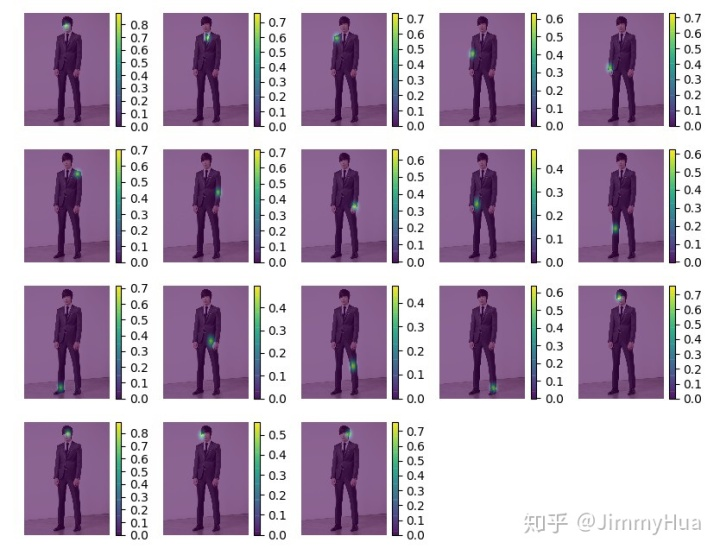- 输出姿态：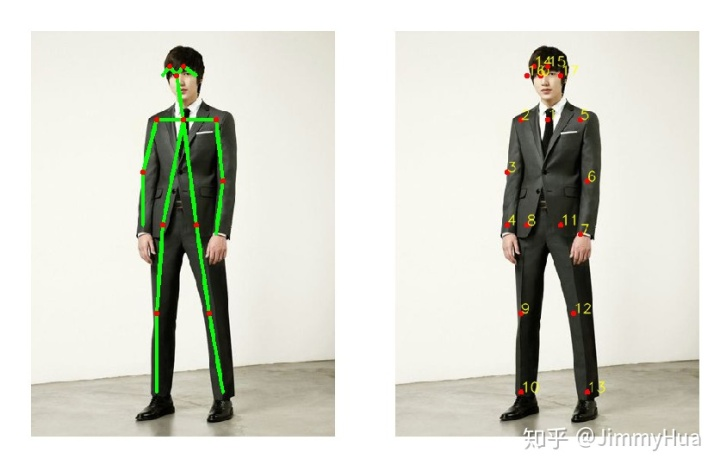------------------------------------------可爱の分割线------------------------------------------更多Opencv教程可以 Follow github的opencv教程，中文&English 欢迎Star❤️❤️❤️JimmyHHua/opencv_tutorials​github.com展开全文• 人脸关键点检测是指给定人脸图像，定位出人脸面部的关键点，包括眉毛、眼睛、鼻子、嘴、脸部轮廓区域的点，由于受到姿态和遮挡等因素的影响，关键点检测是一个富有挑战性的任务。 人脸关键点有以下主要的应用： ...人脸识别 深度学习 人工智能 计算机视觉
• 本文讲的是什么是云原生应用 有哪些关键点？【IT168 评论】最近讨论云原生应用越来越多，其是指原生为在云平台上部署运行而设计开发的应用。公平的说，大多数传统的应用，不做任何改动，都是可以在云平台运行起来的...
• 人脸关键点检测是诸如人脸识别、表情分析、三维人脸重建等其它人脸相关任务的基础。近些年来，深度学习方法已被成功应用到了人脸的关键点检测，本章将介绍...人脸关键点是人脸各个部位的重要特征点，通常是轮廓点与角
• 特征点是什么 SLAM需要根据路标来计算当前相机的位置和姿态。而视觉SLAM的路标就是图像中的特征点了。...关键点指的特征点在图像中的位置，而描述子是指的是关键点的朝向和周围像素信息。相同特征...
• 一、什么是关键点位？ 本交易策略的关键点位通常日线级别形成强阻力支撑或突破的行为重要价格位置。比如关键K线（大阳线、大阴线）位置、正N突破波峰位置、头肩底颈线位置、W底颈线位置、上升三角形水平阻力线...股票 日内交易
• PLM系统的实施是指在PLM框架下实现企业最佳运作(主要指产品开发)的过程。它根据企业需求和企业文化，将所有与产品相关的信息、资源、人员和过程都纳入PLM技术和管理框架之中，实现优化运作。 它的实施解决了集成...
• OpenCV实现人体姿态估计(人体关键点检测）OpenPose OpenPose人体姿态识别项目美国卡耐基梅隆大学（CMU）基于卷积神经网络和监督学习并以Caffe为框架开发的开源库。可以实现人体动作、面部表情、手指运动等姿态...人体姿态估计 OpenPose
• 原文：OpenPose 基于OpenCV DNN 的手部关键点检测 - AIUAI 手部关键点检测，旨在找出给定图片中手指上的关节点及指尖关节点. 其类似于面部关键点检测(Facial Landmark Detection) 和人体关键点检测(Human Body Pose ...openpose
• 人脸关键点定位也称为人脸关键点检测，是指给定人脸图像，定位出人脸面部的一些关键位置，包括人脸轮廓及五官等。是 3D 人脸重建、表情迁移、特效相机等应用的核心基础技术。 为助力疫情期间戴口罩场景下的关键点...深度学习 人脸识别 计算机视觉
• 前言总结了Java异常十个关键知识，面试或者工作中都有用哦，加油。一. 异常是什么异常是指阻止当前方法或作用域继续执行的问题。比如你读取的文件不存在，数组越界，进行除法时，除数为0等都会导致异常。一个文件...
• 一只Tom猫：三次阿里二面... 异常是什么异常是指阻止当前方法或作用域继续执行的问题。比如你读取的文件不存在，数组越界，进行除法时，除数为0等都会导致异常。一个文件找不到的异常：public class TestException {...java 抛出异常
• 1.信息门户的定义 什么是门户？...在协同OA系统领域，所谓信息门户（Information Portal）是指利用网络浏览器访问组织内部和外部关键信息的统一入口，可以针对每个用户进行个性化设置。它的作用是解决...协同OA系统
• 前言总结了Java异常十个关键知识，面试或者工作中都有用哦，加油。一. 异常是什么异常是指阻止当前方法或作用域继续执行的问题。比如你读取的文件不存在，数组越界，进行除法时，除数为0等都...
• 集成华为手部关键点识别服务轻松识别手语字母 介绍 华为机器学习（ML Kit）提供手部关键点识别服务，可用于手语识别。手部关键点识别服务能识别手部21个关键点，通过每个手指的方向和手语规则作比较去找手语字母表。...android
• 现在人们手上最少都有一部手机，而且很多人都直接两部手机... 关键点一、手机类型手机类型其实就是的分辨率问题，而且这个问题早就已经在视频制作行业里面发生了，因为以前大部分的电视分辨率都四比三的，但...手机网站建设
• Offer——京东校招笔试题+知识总结笔试感言 经过一系列的笔试，发觉自己的基础知识还是比较薄弱的，尤其数据结构和网络，还有操作系统。工作量还是很大的。做到精确制导的好方法就是在网上刷题，包括牛客和...笔试
• Offer——迅雷笔试题+知识总结情景回顾时间:2016.9.19 19：00-21：00地点:山东省网络环境智能计算技术重点实验室事件:迅雷笔试 总体来说，迅雷笔试内容体量不算多，主要分为30道选择题，2道编程题，半小时将...堆栈...